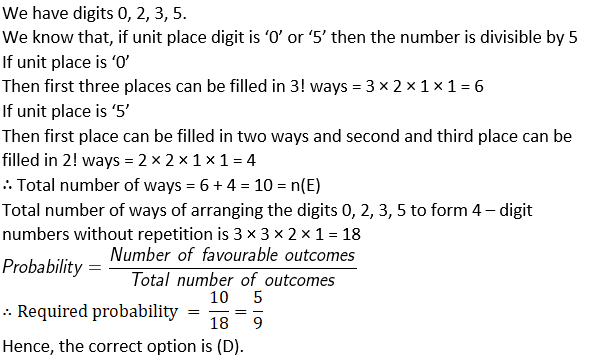# NCERT Exemplar Solutions for Class 11 Maths Chapter 16 Probability

NCERT Exemplar Solutions for Class 11 Maths Chapter 16 Probability is presented here for the benefit of the students preparing for the examination. It is very important for the students to get well versed with these solutions to get a good score in Class 11 examination. These NCERT Exemplar problems are solved by experts at BYJU’S. These solutions will help you understand and master different types of questions on probability. NCERT Exemplar solutions help you to attain perfection in solving different kinds of questions.

The PDF of NCERT Exemplar Solutions for Class 11 Maths Chapter 16 Probability is provided here. Students can refer and download from the given links. These solutions facilitate students to create good knowledge about basic concepts of Mathematics. This chapter is based on random experiments. Some of the essential topics of this chapter are listed below.

• Random experiment
• Outcome
• Sample Space
• Event
• Types of events
• Impossible and Sure Events
• Simple or Elementary Event
• Compound Event
• Mutually exclusive event
• Exhaustive events
• Axiomatic approach to probability
• Probabilities of equally likely outcomes
• Addition rule for mutually exclusive event

## Download the PDF of NCERT Exemplar Solutions For Class 11 Maths Chapter 16 Probability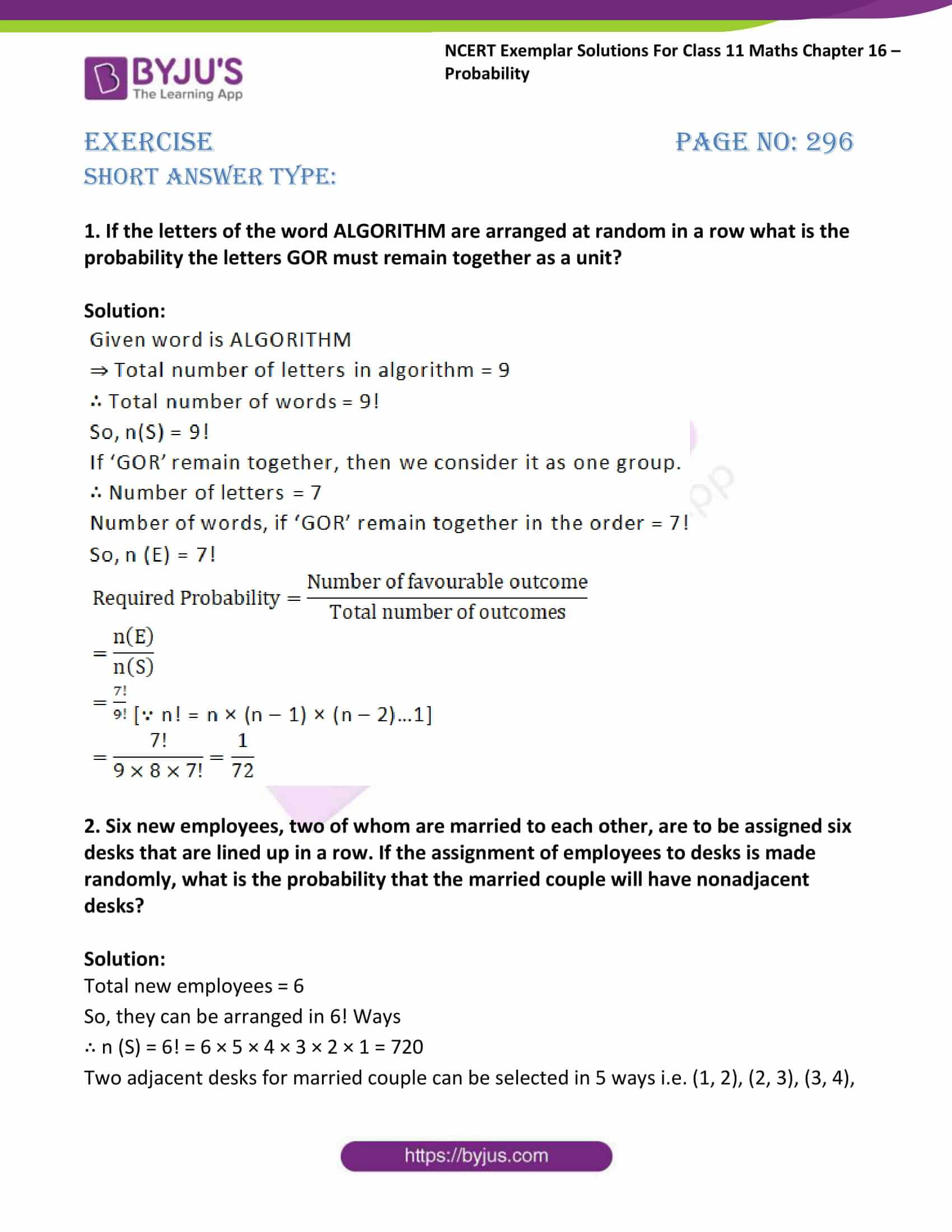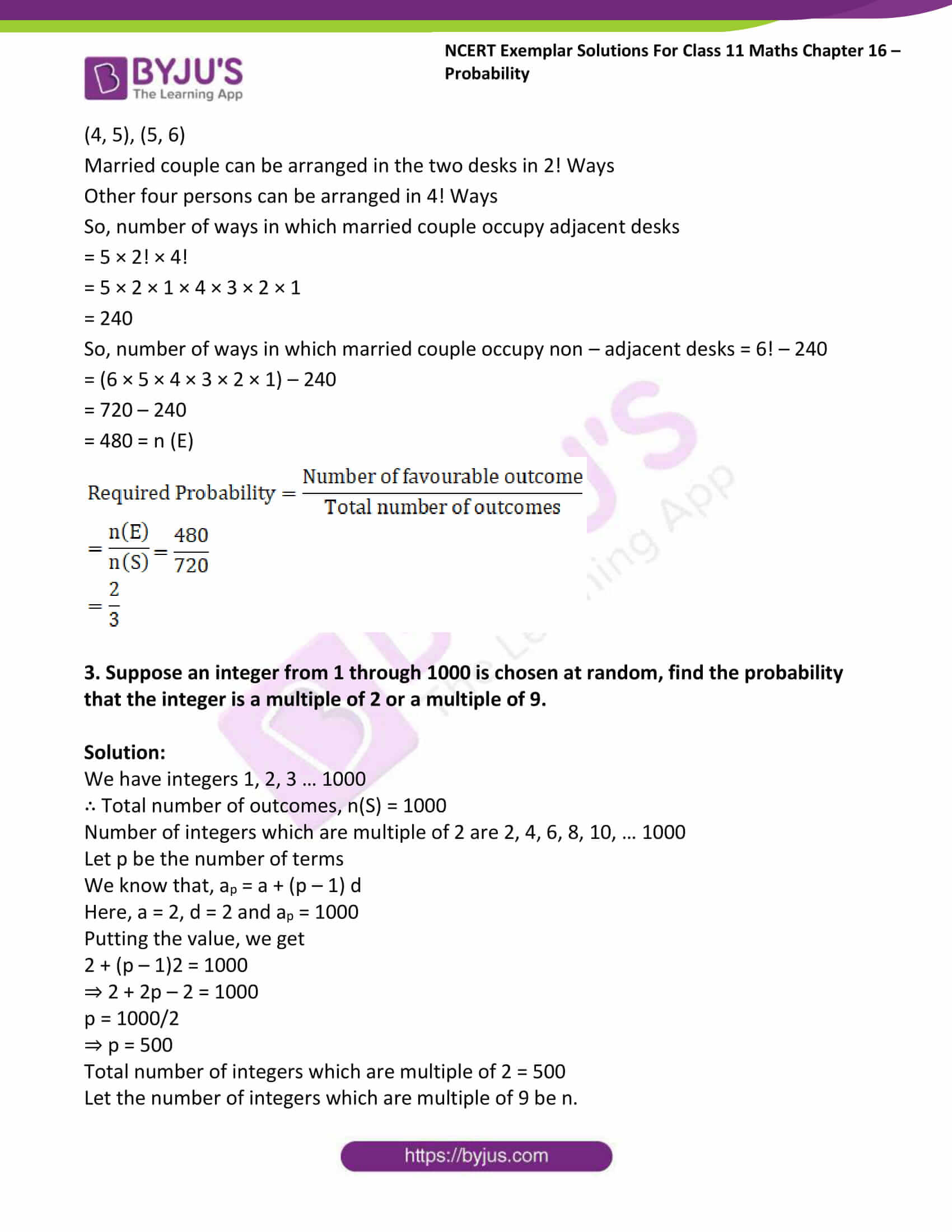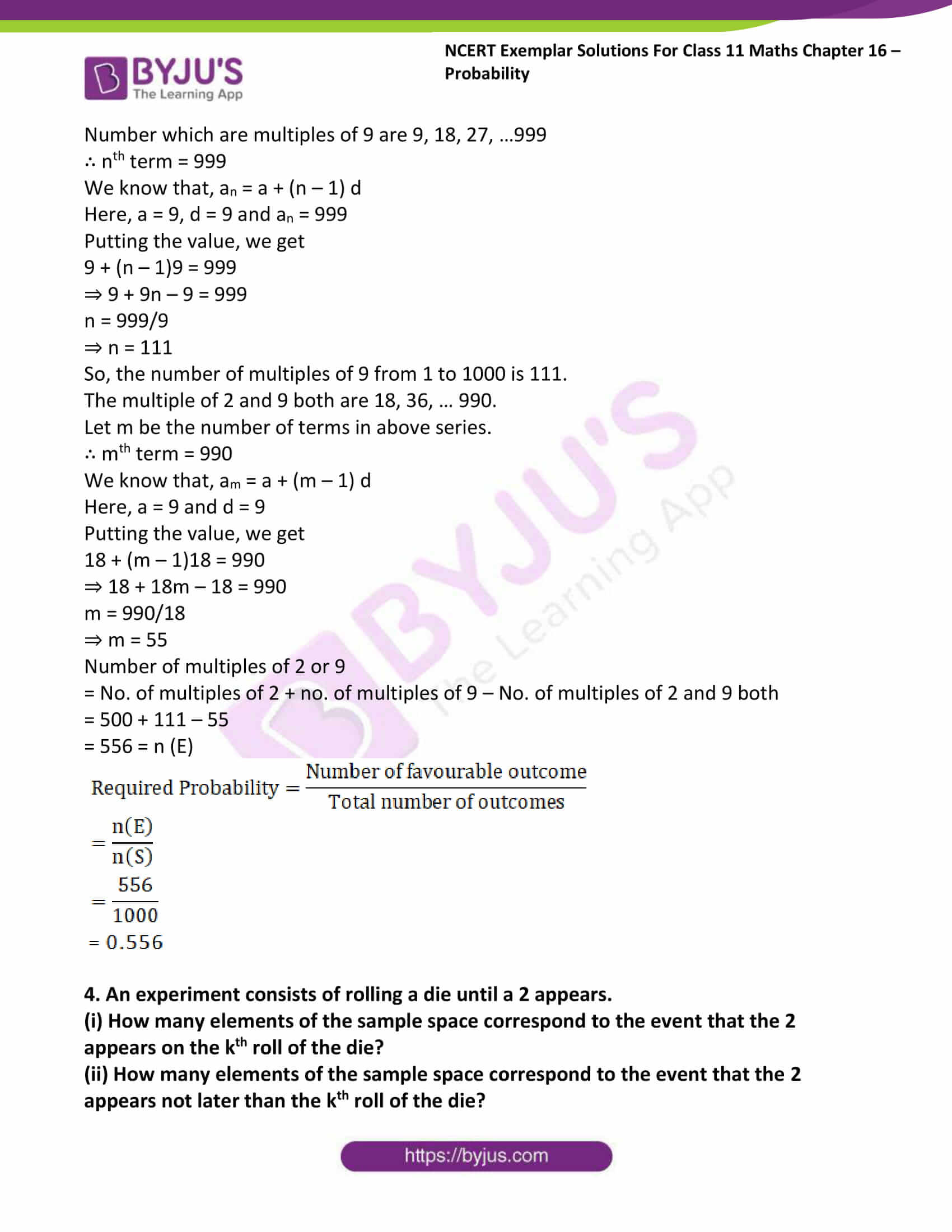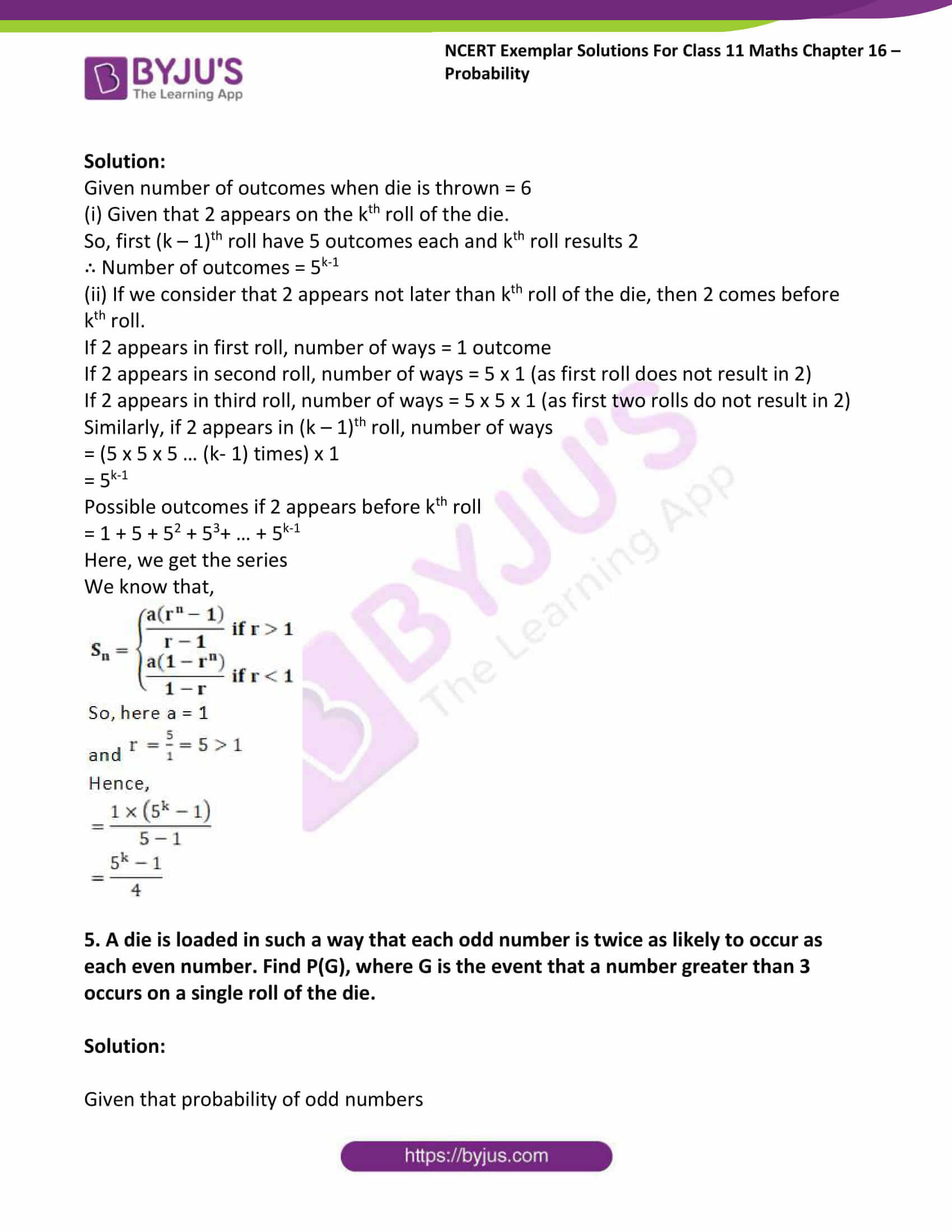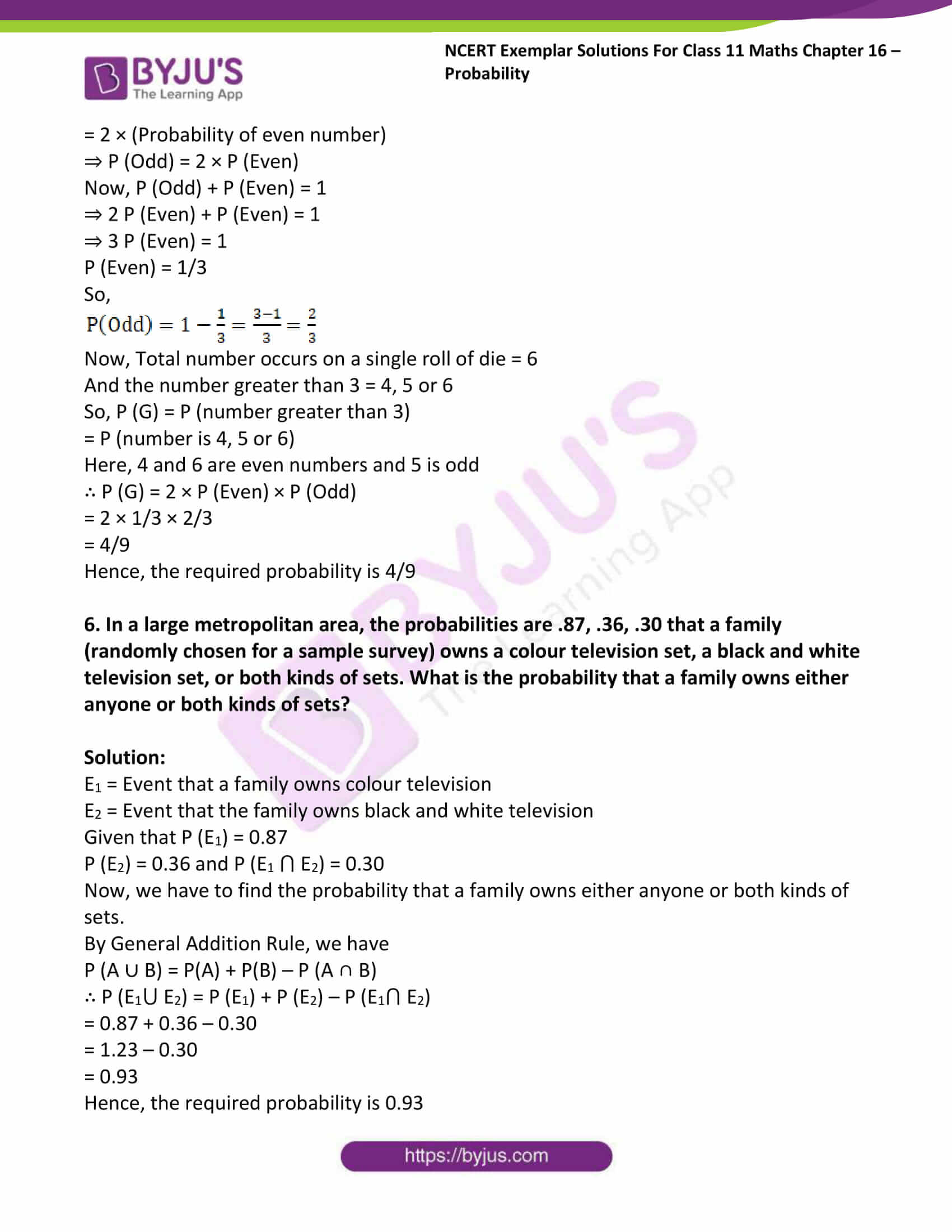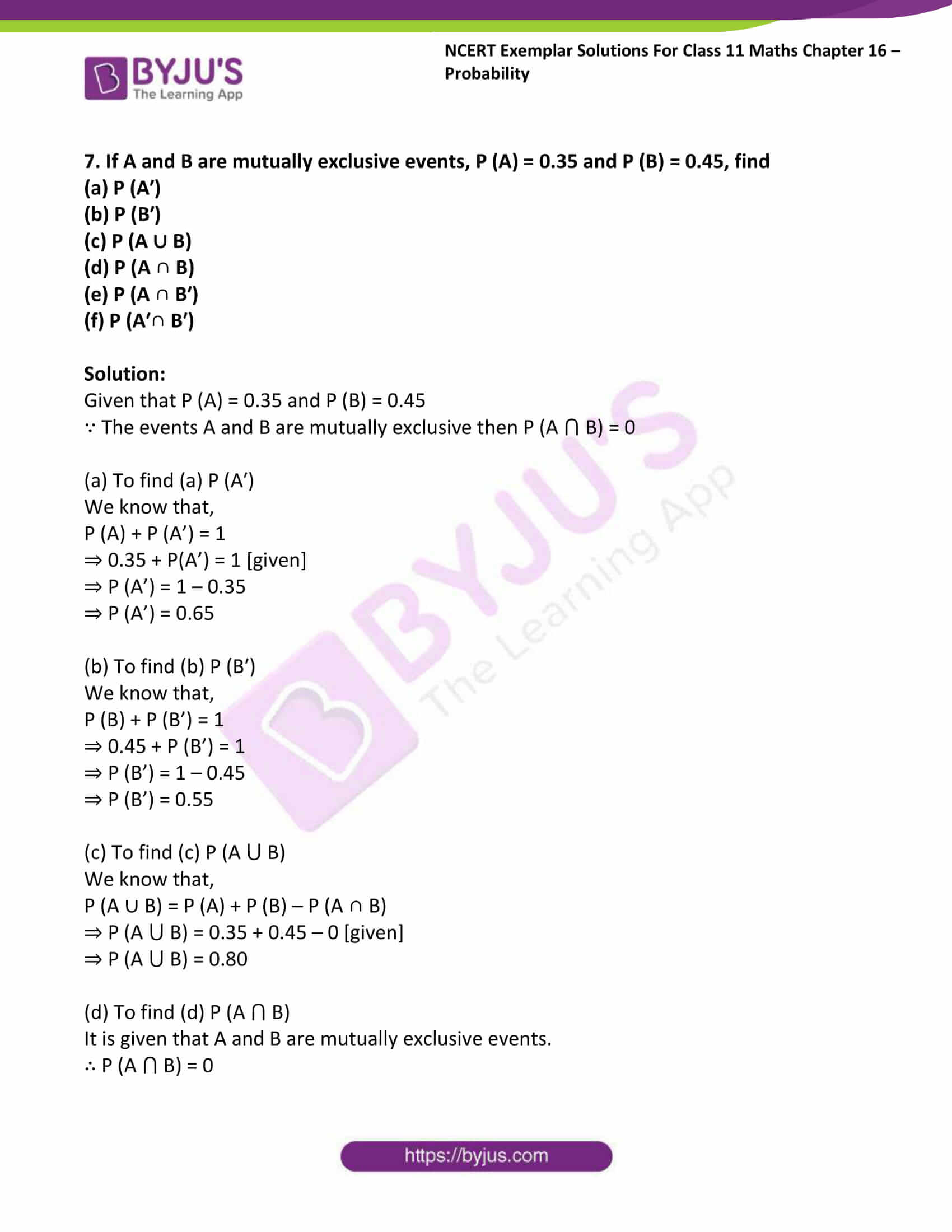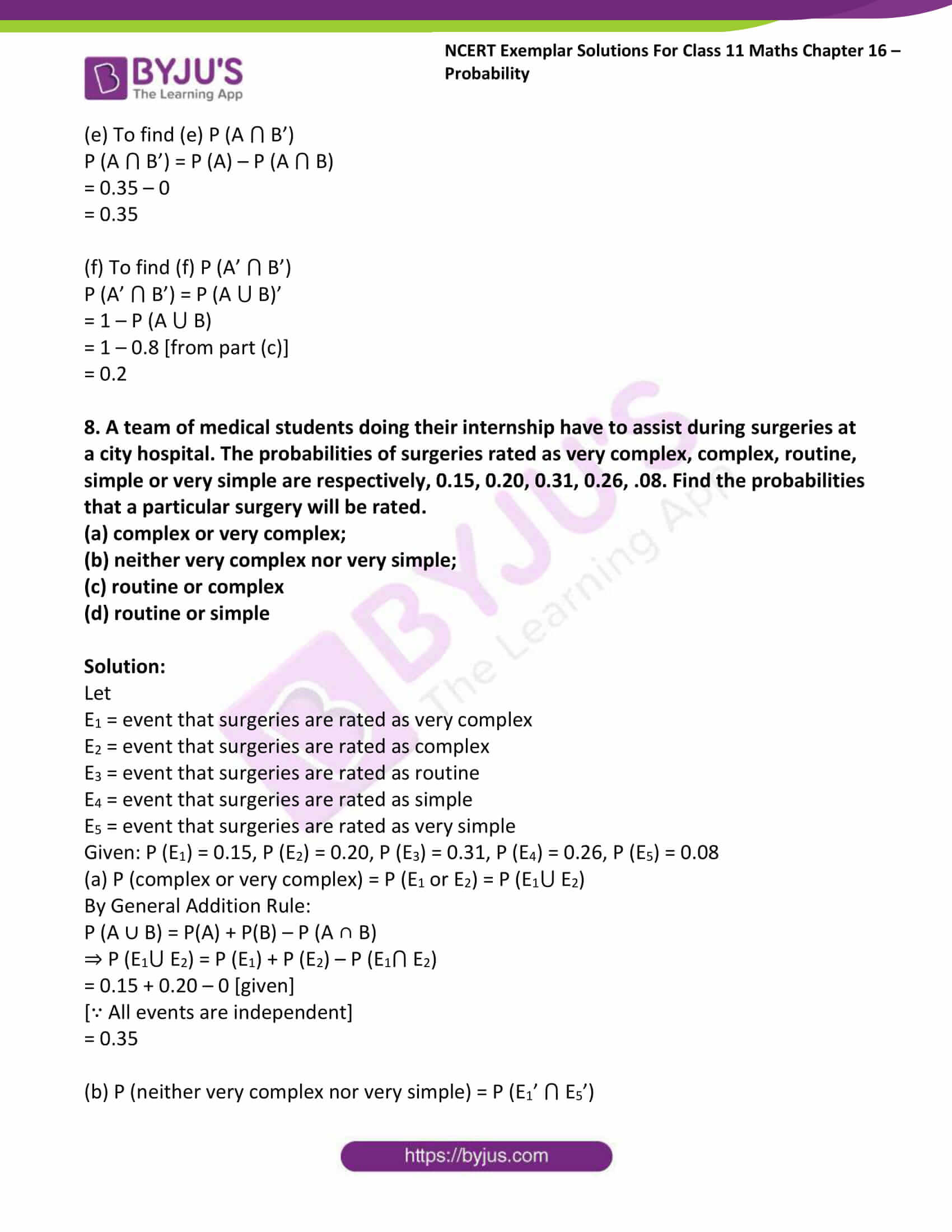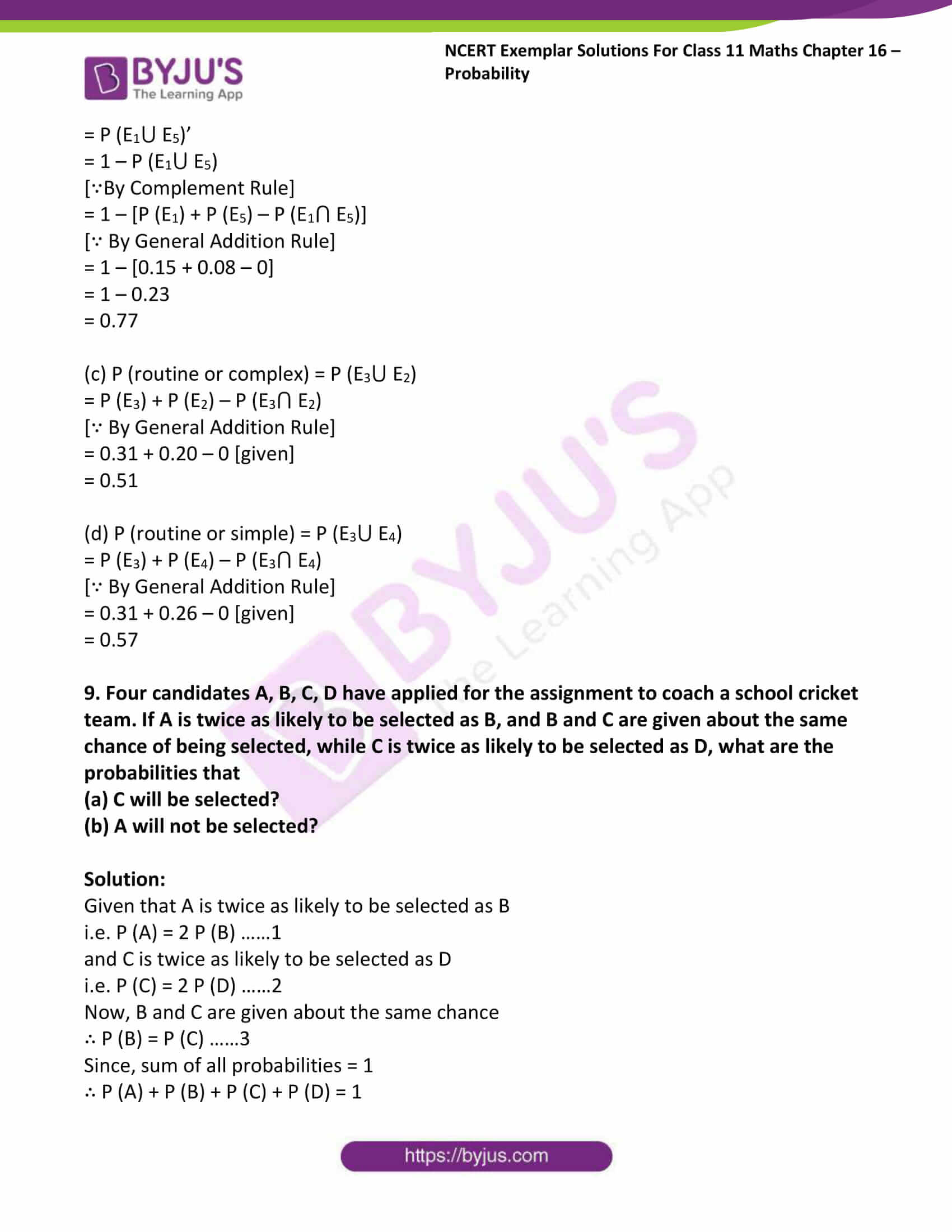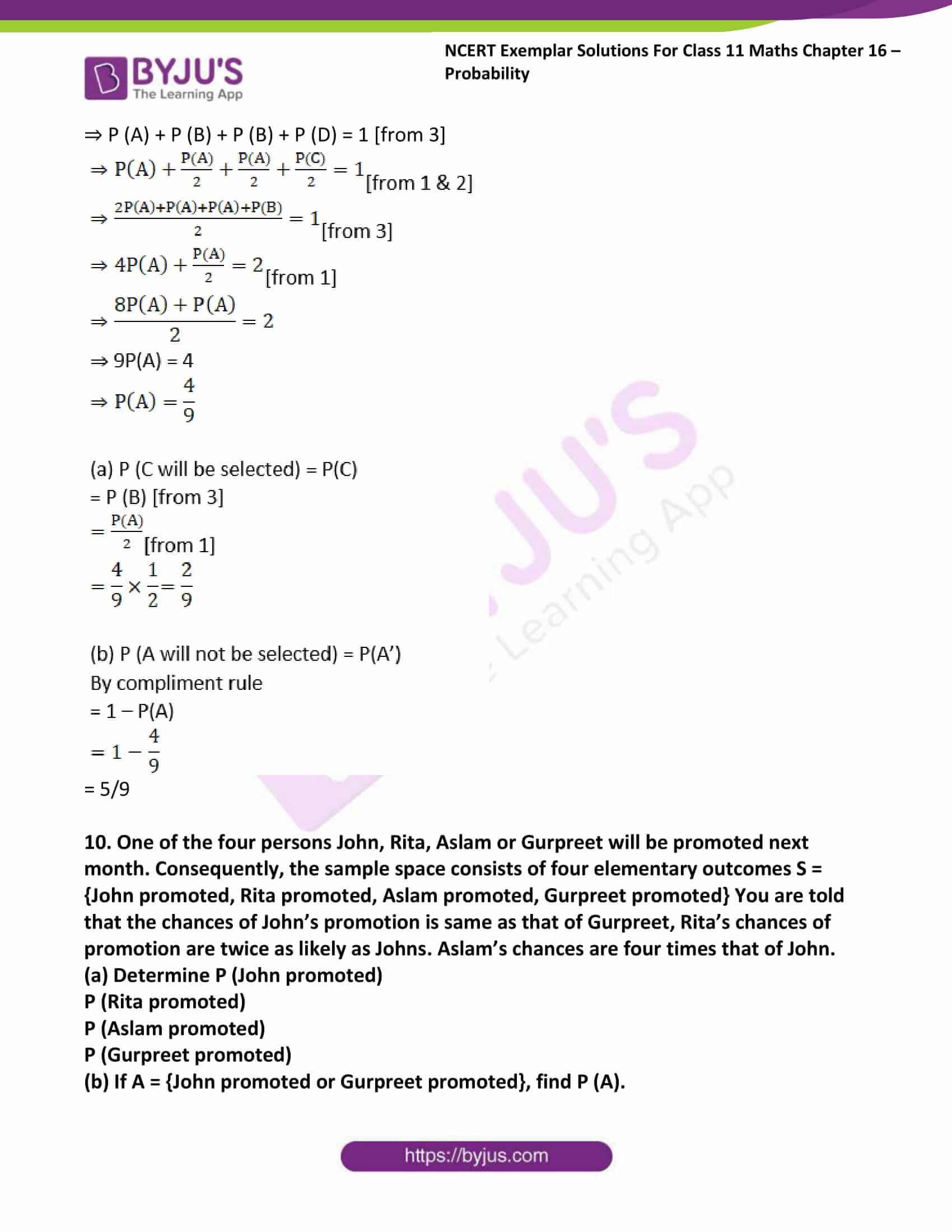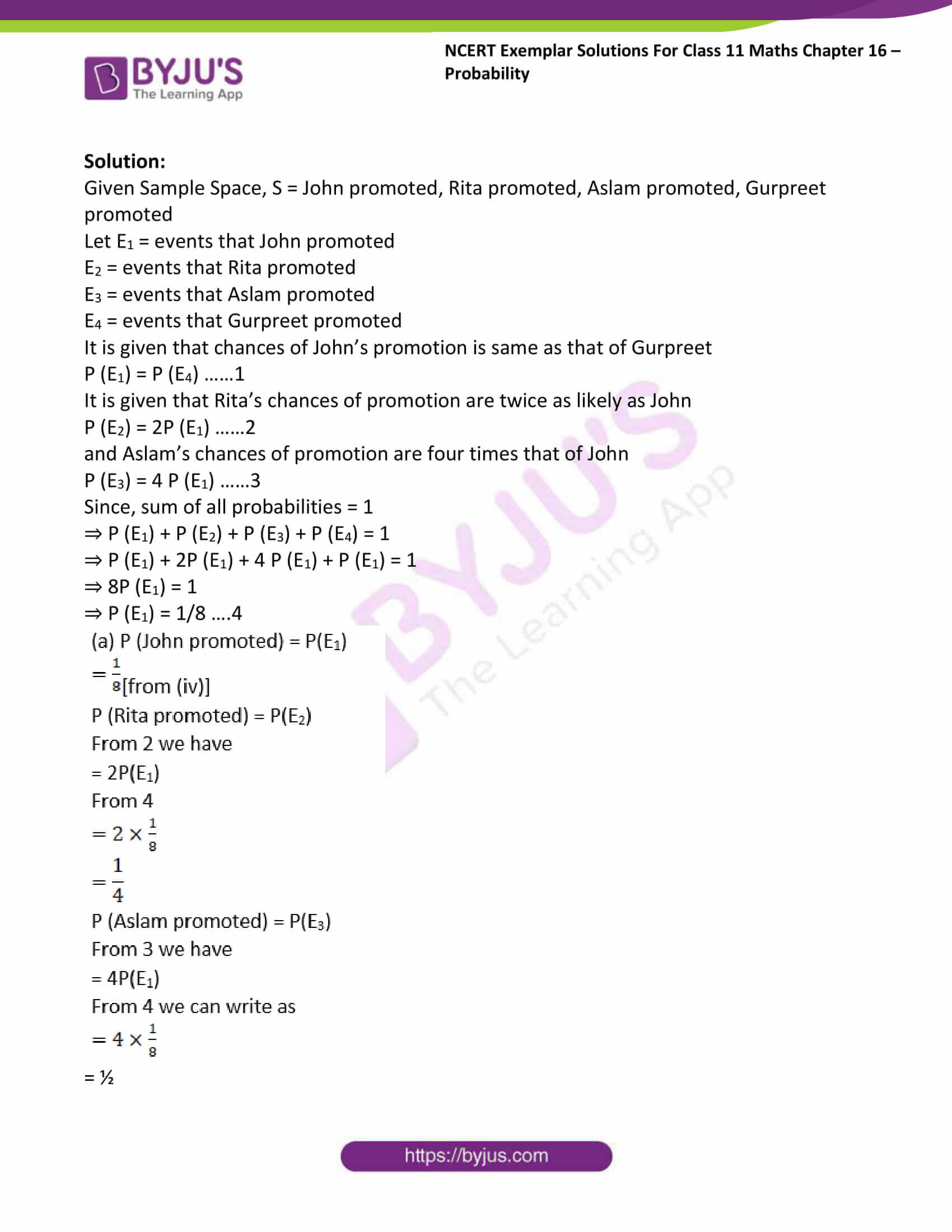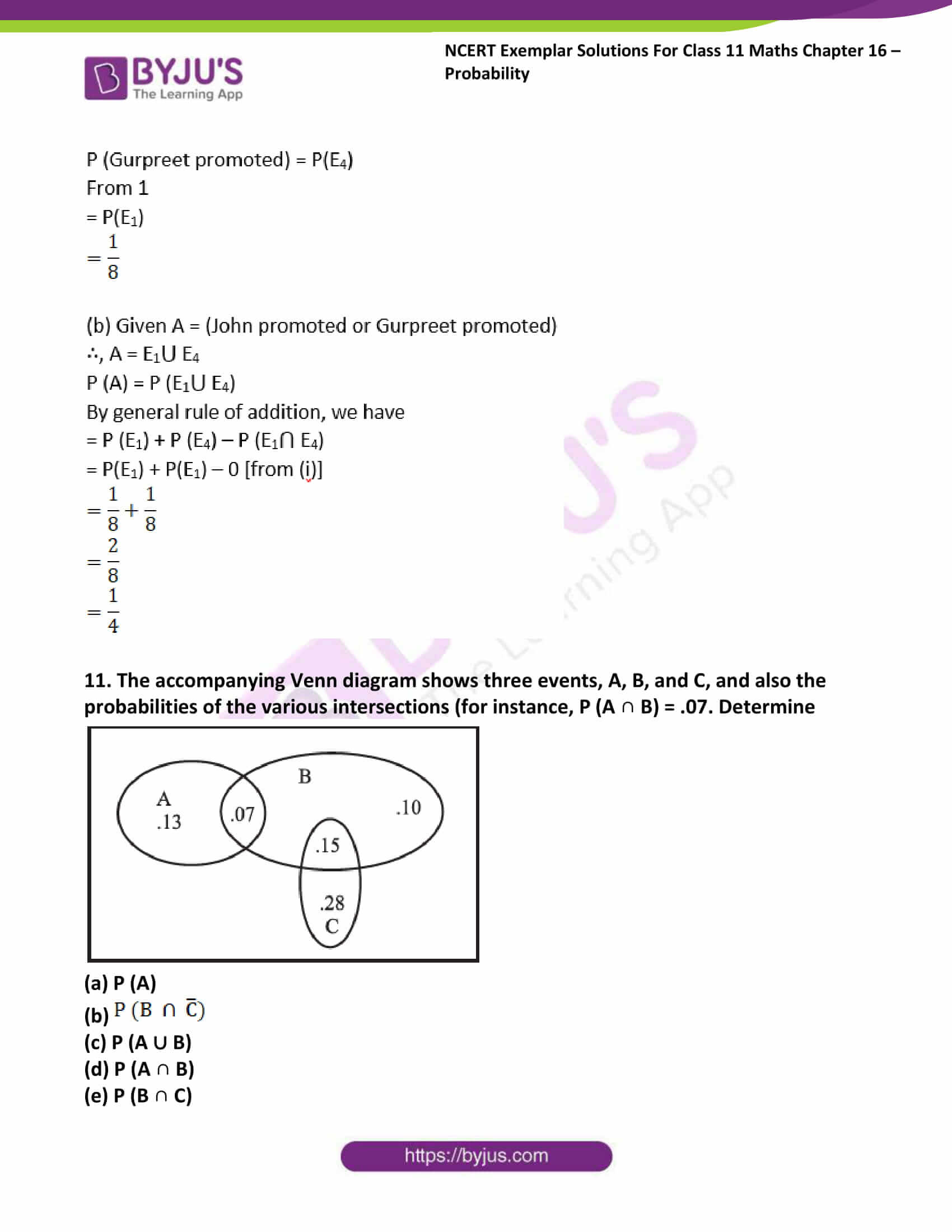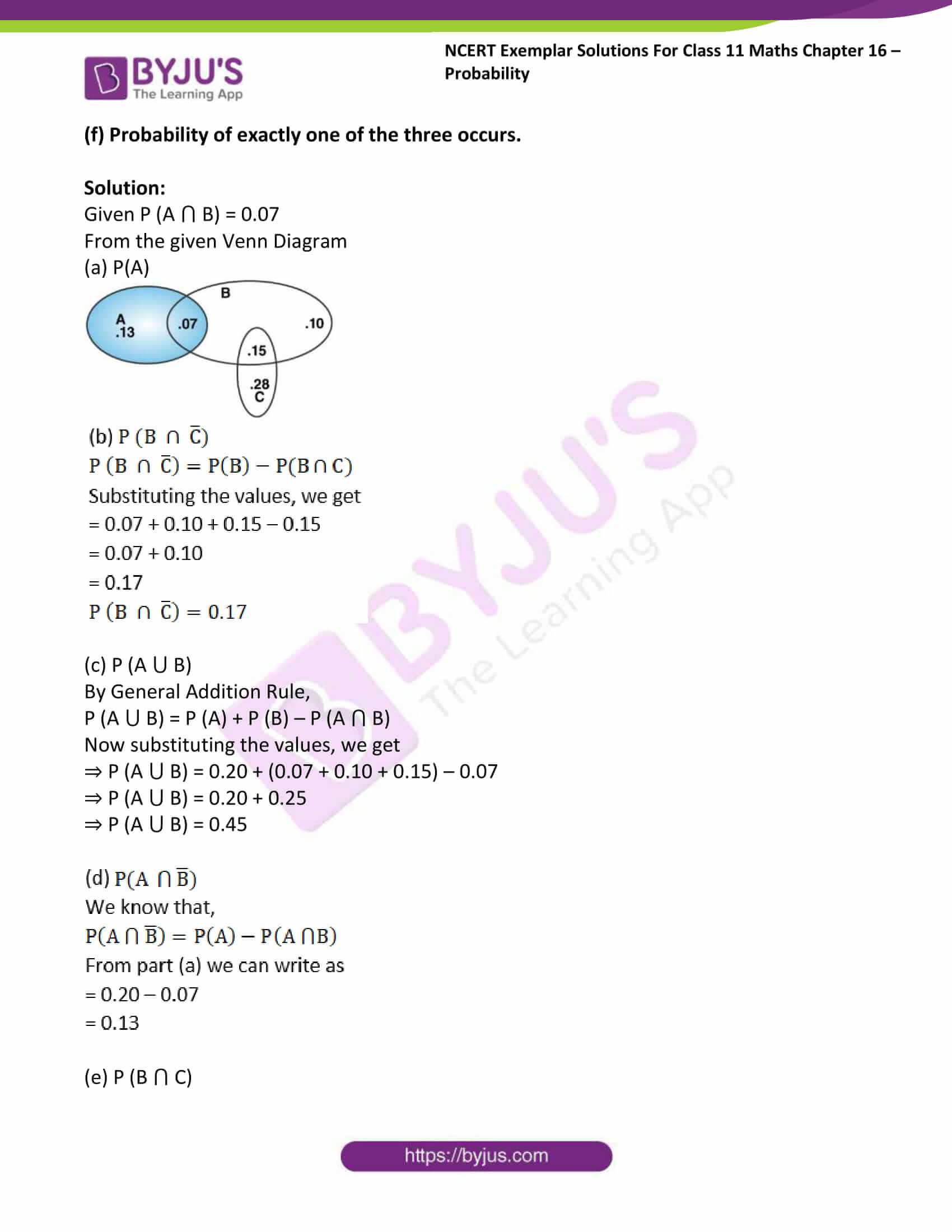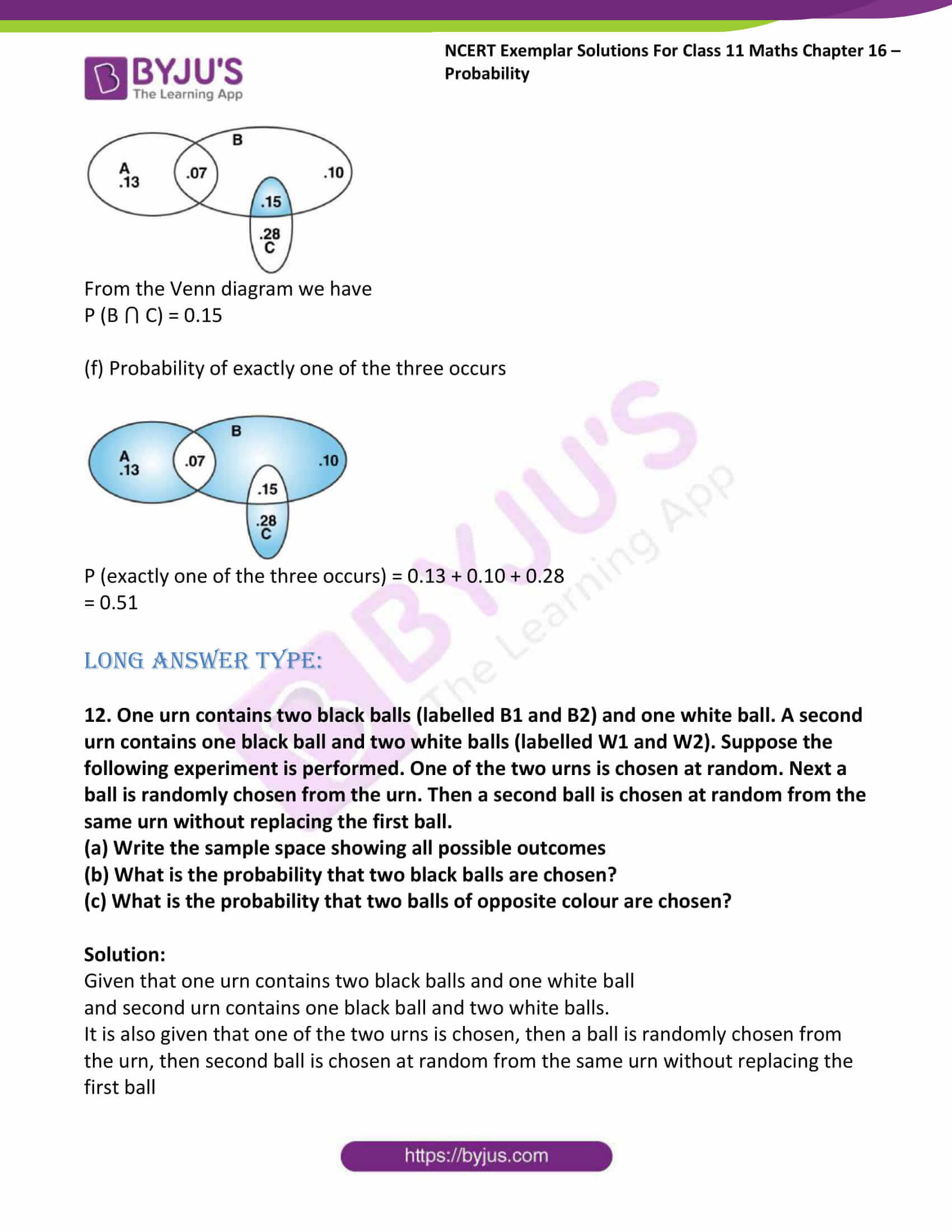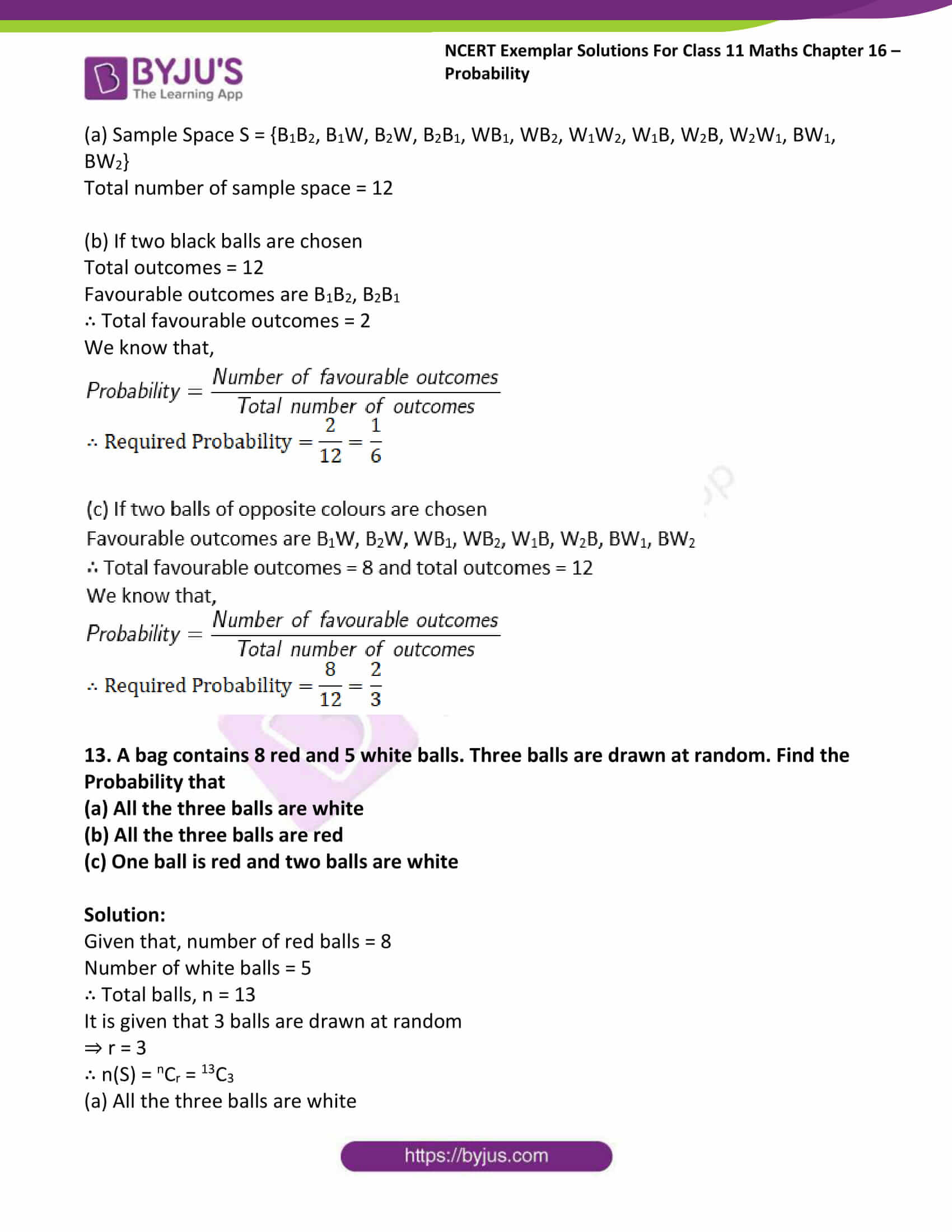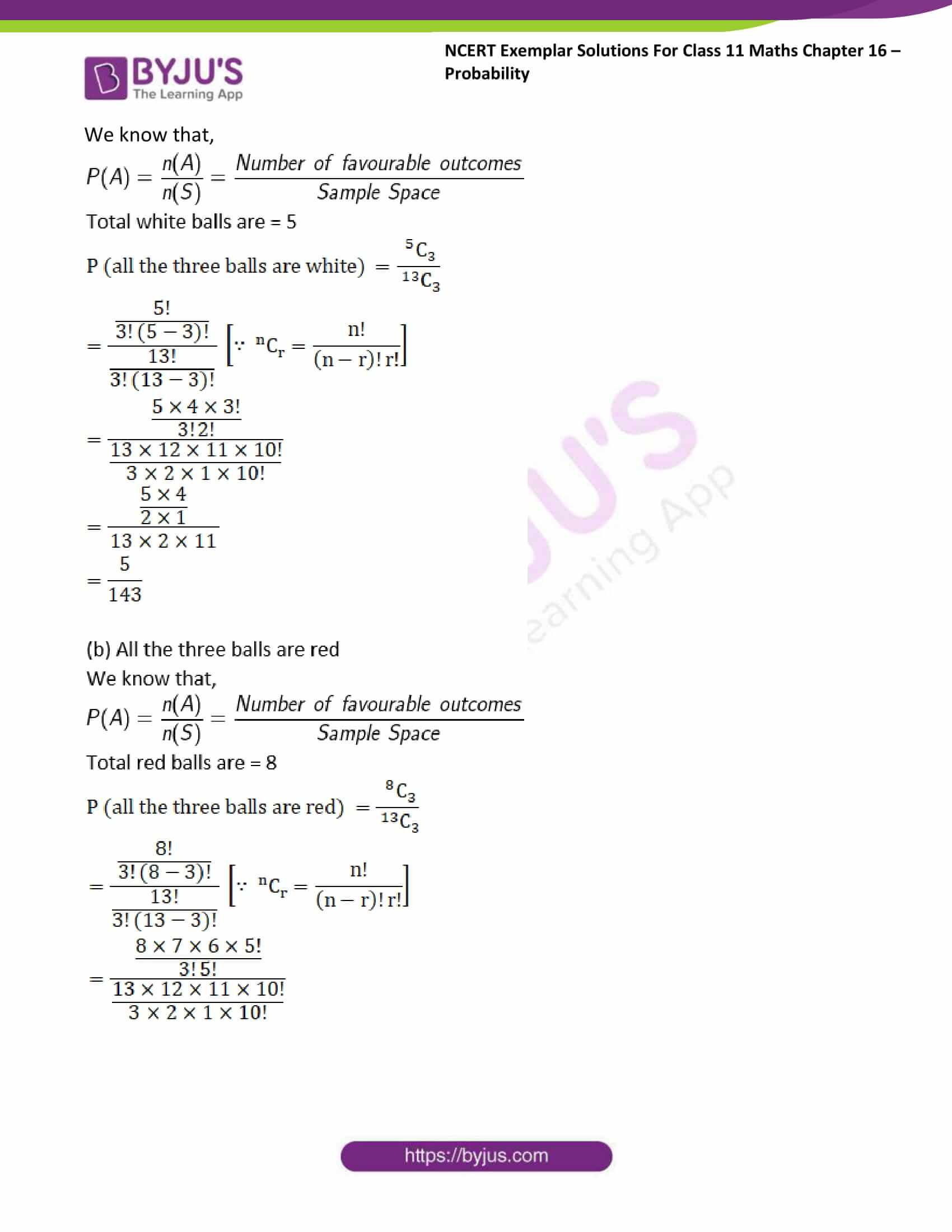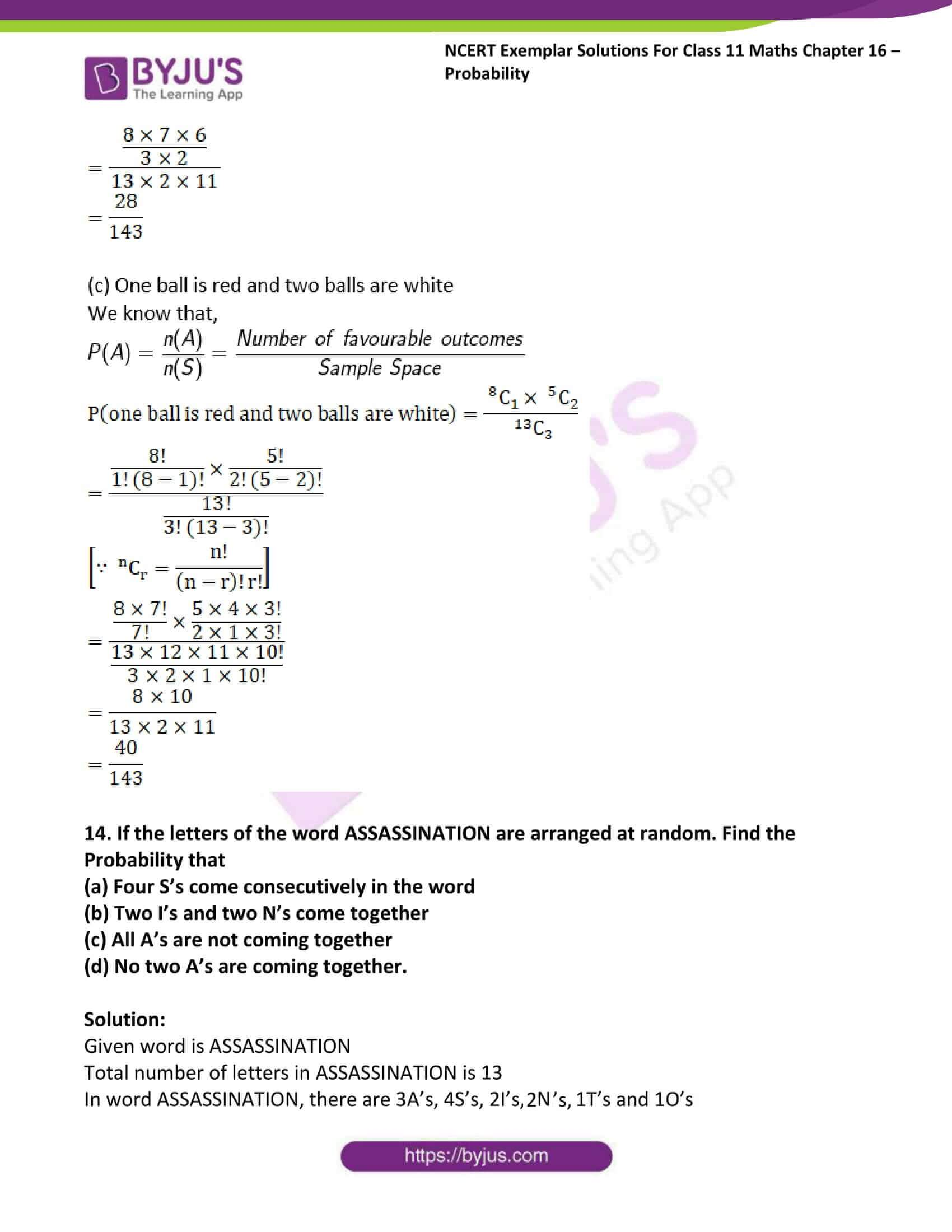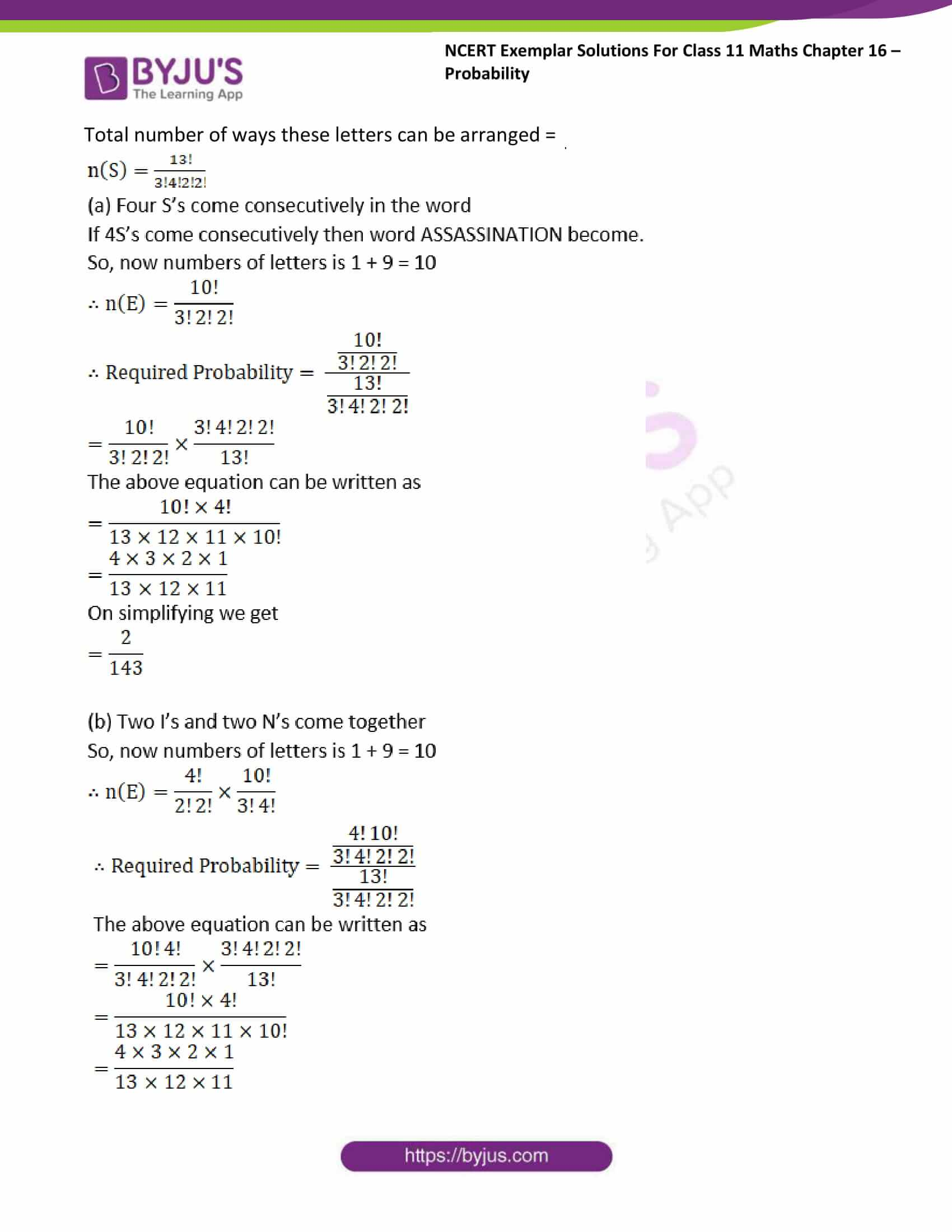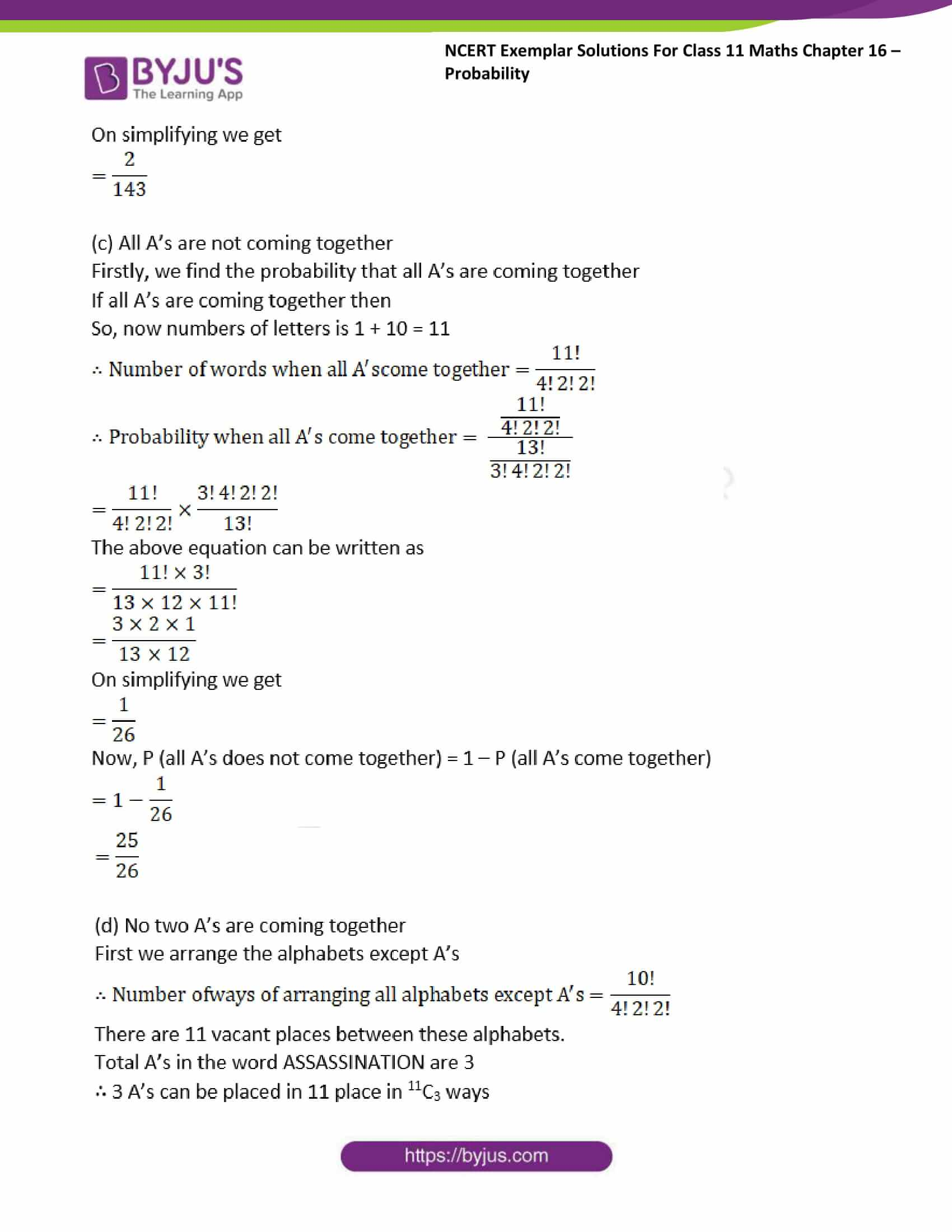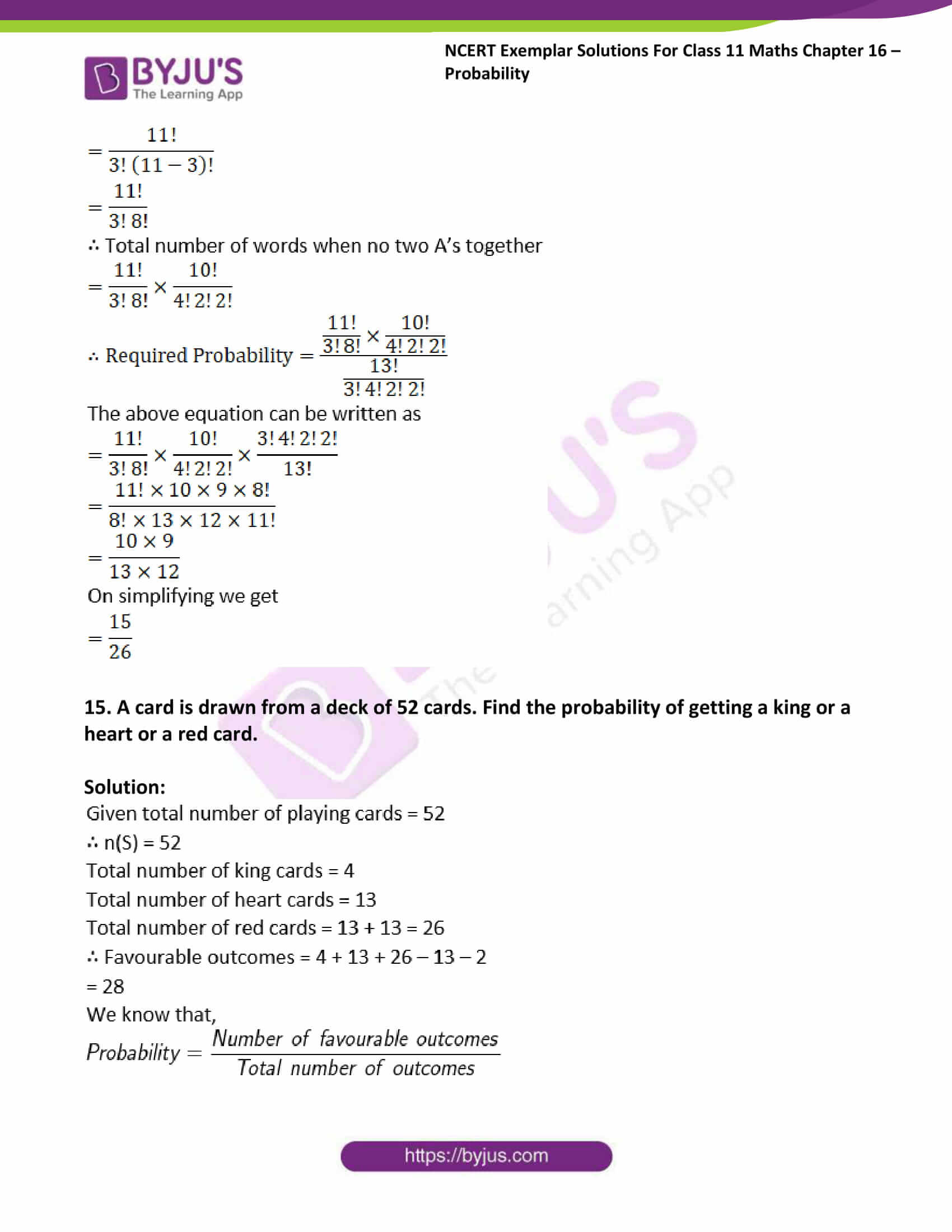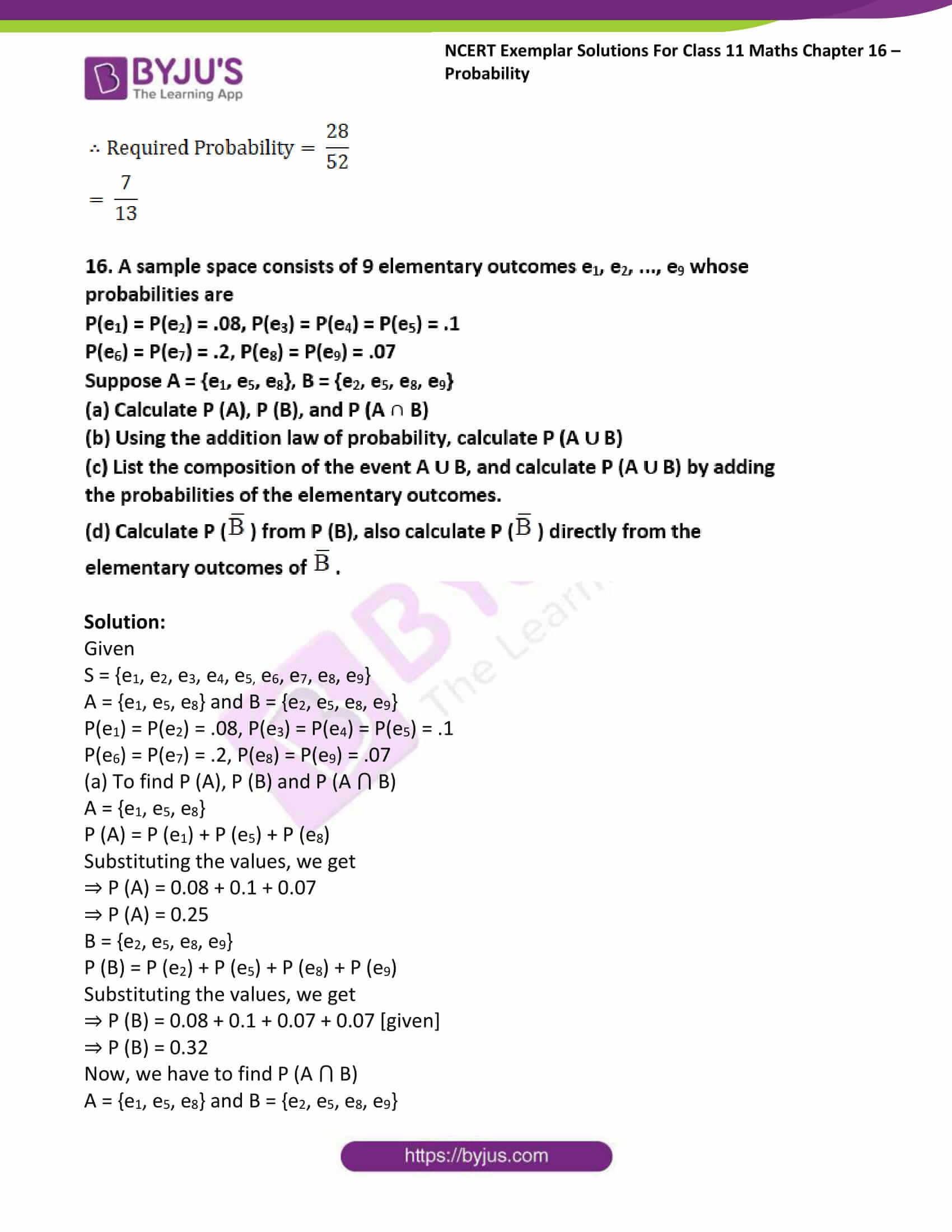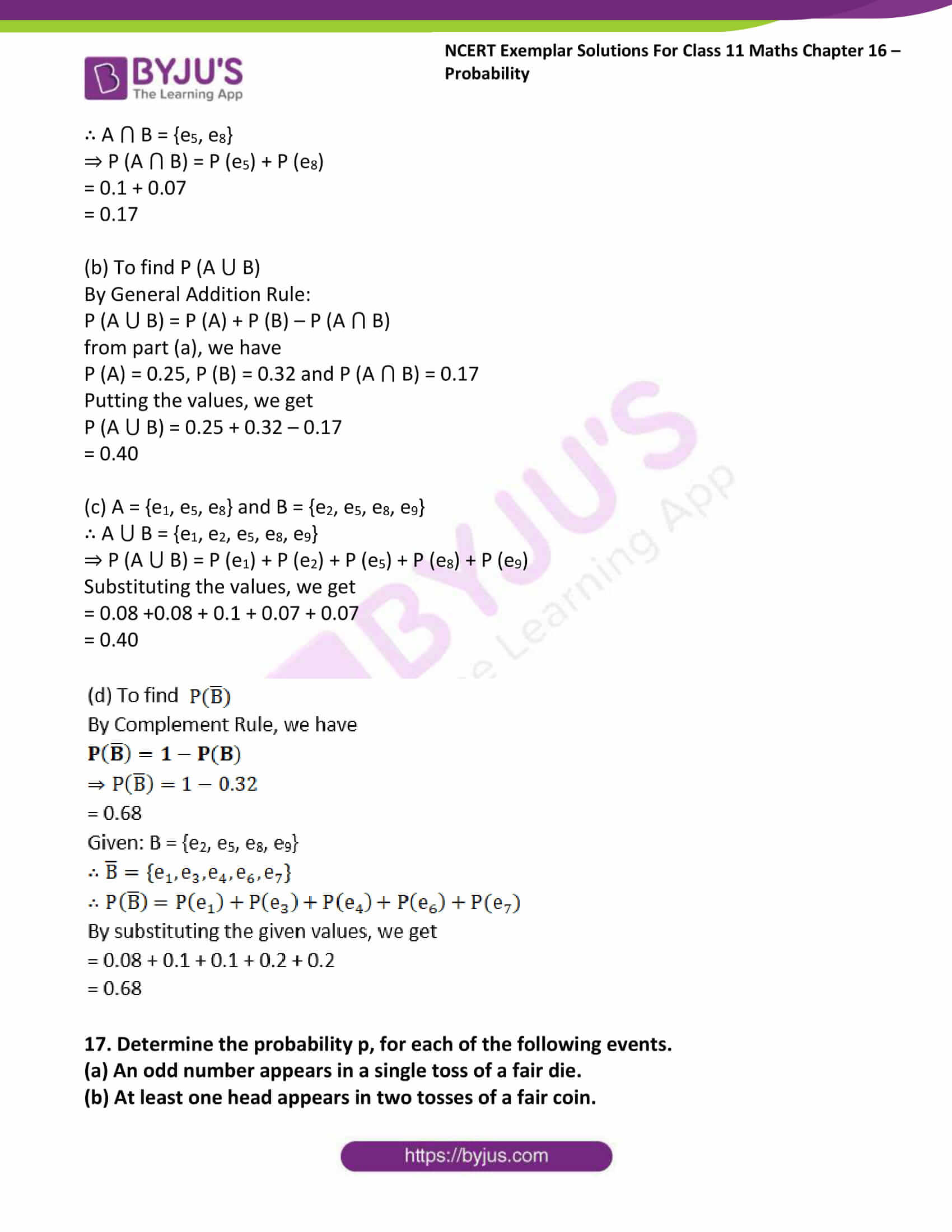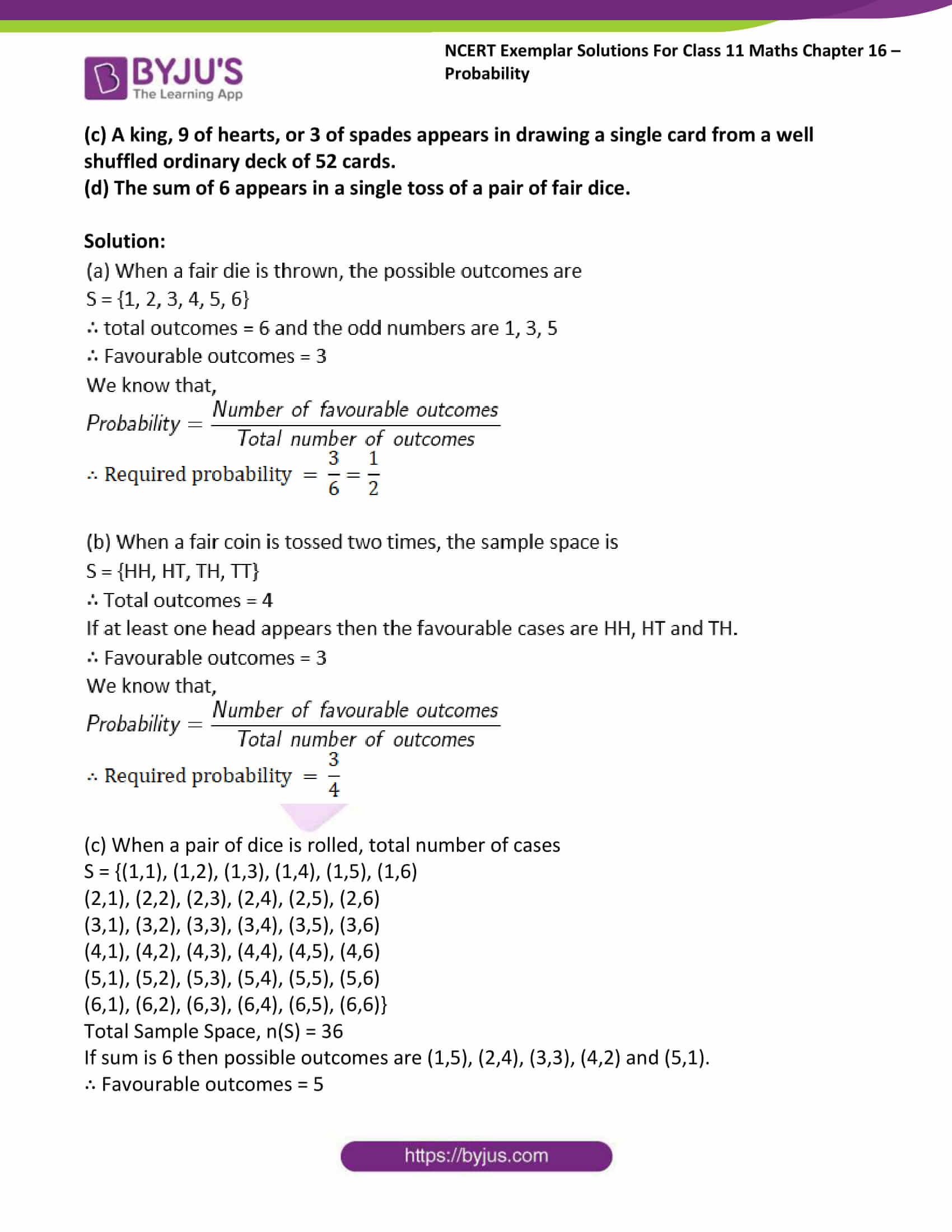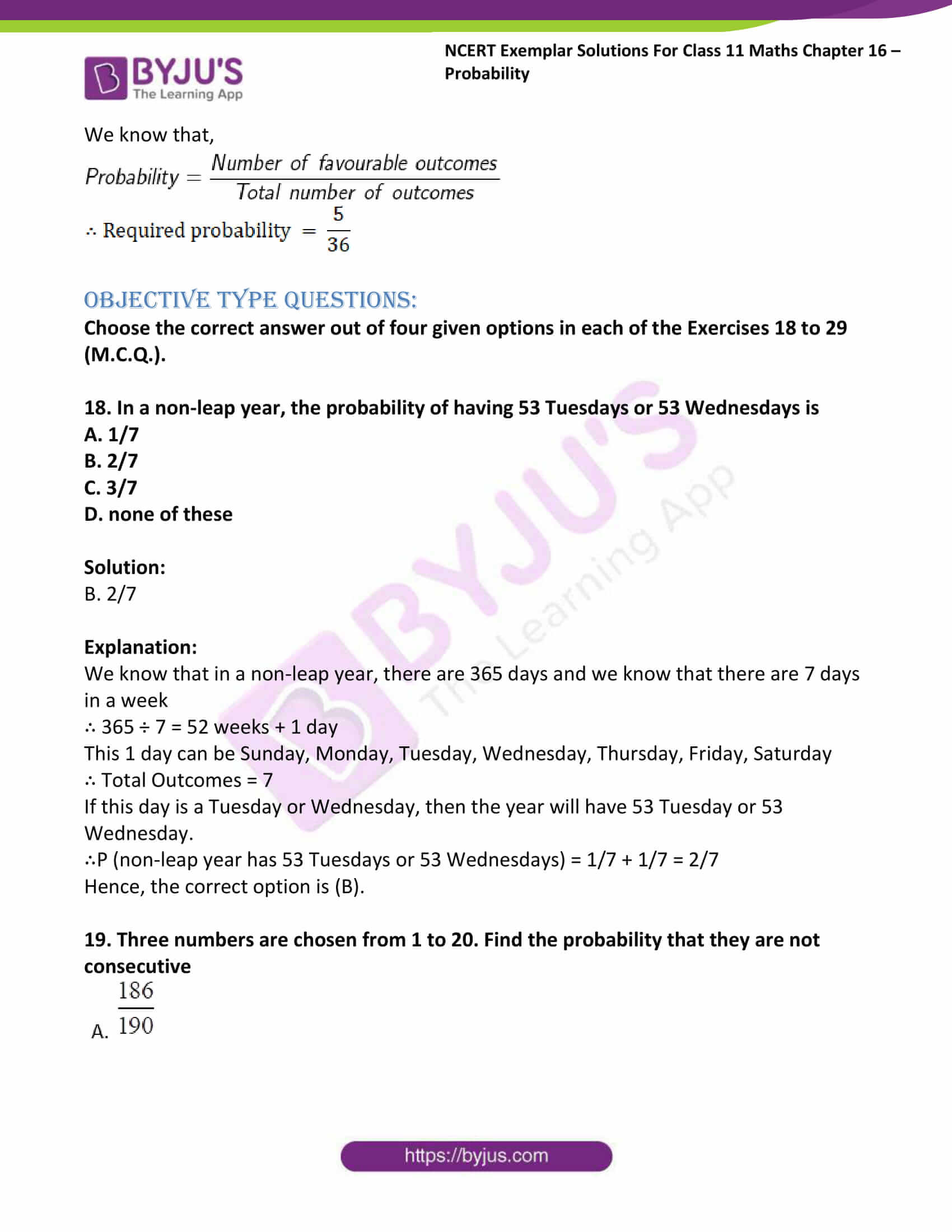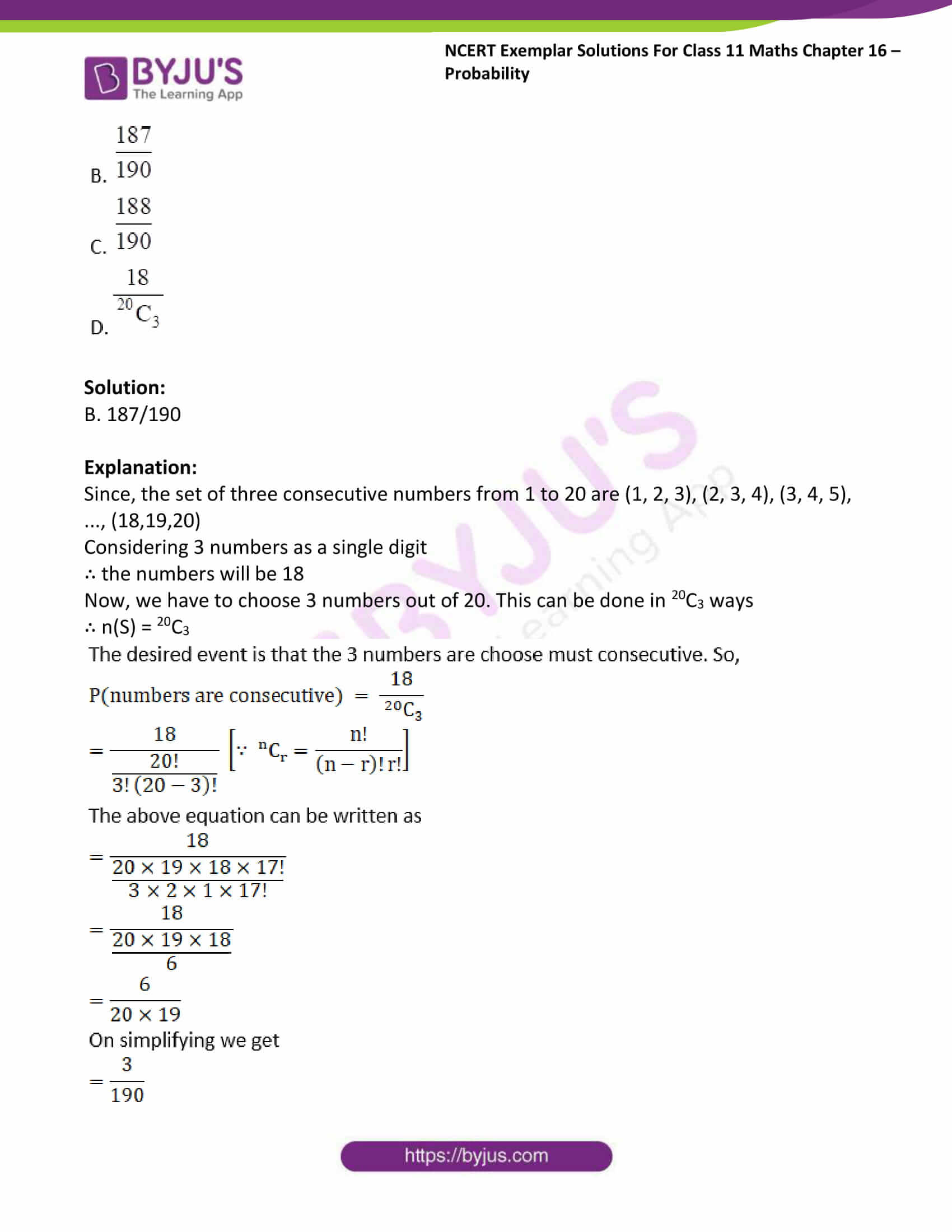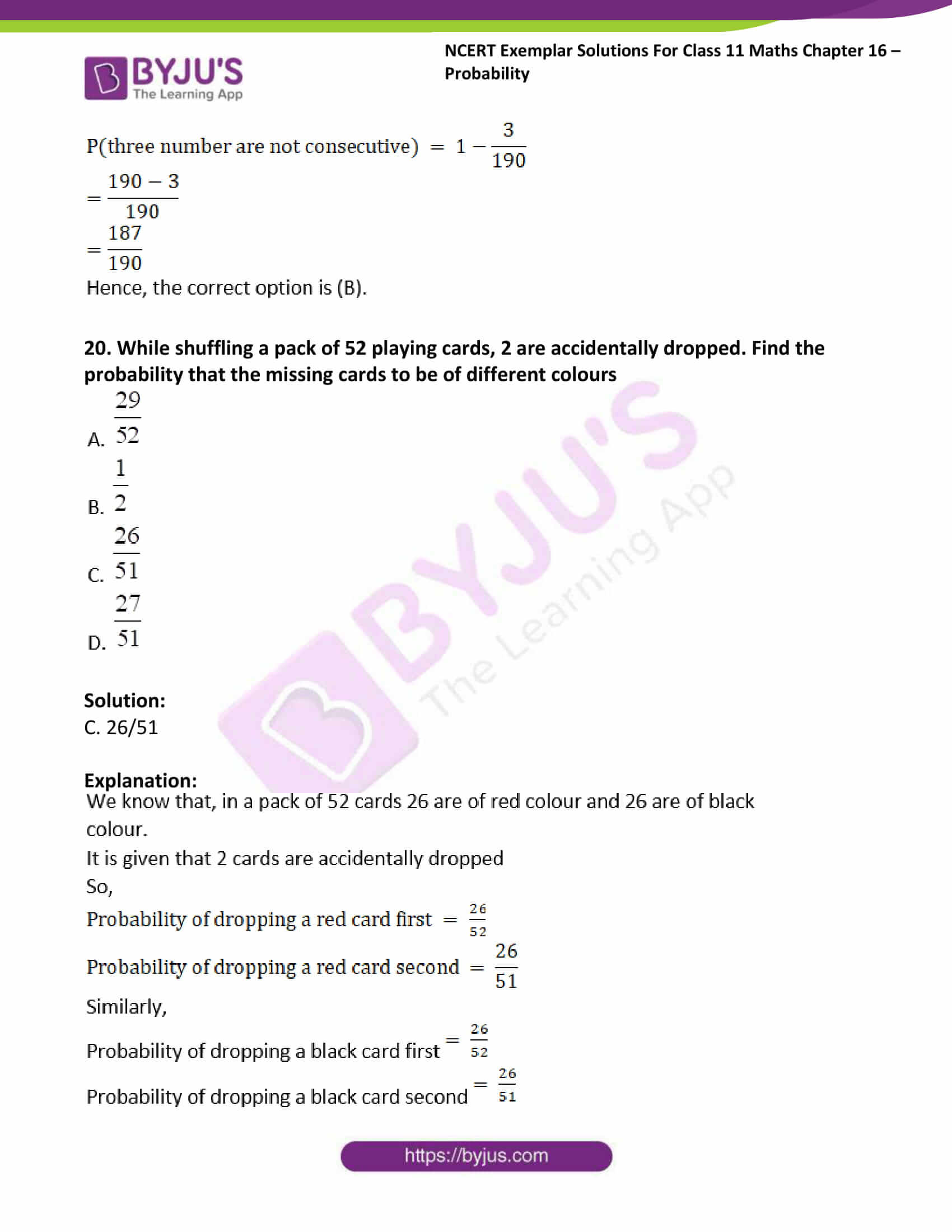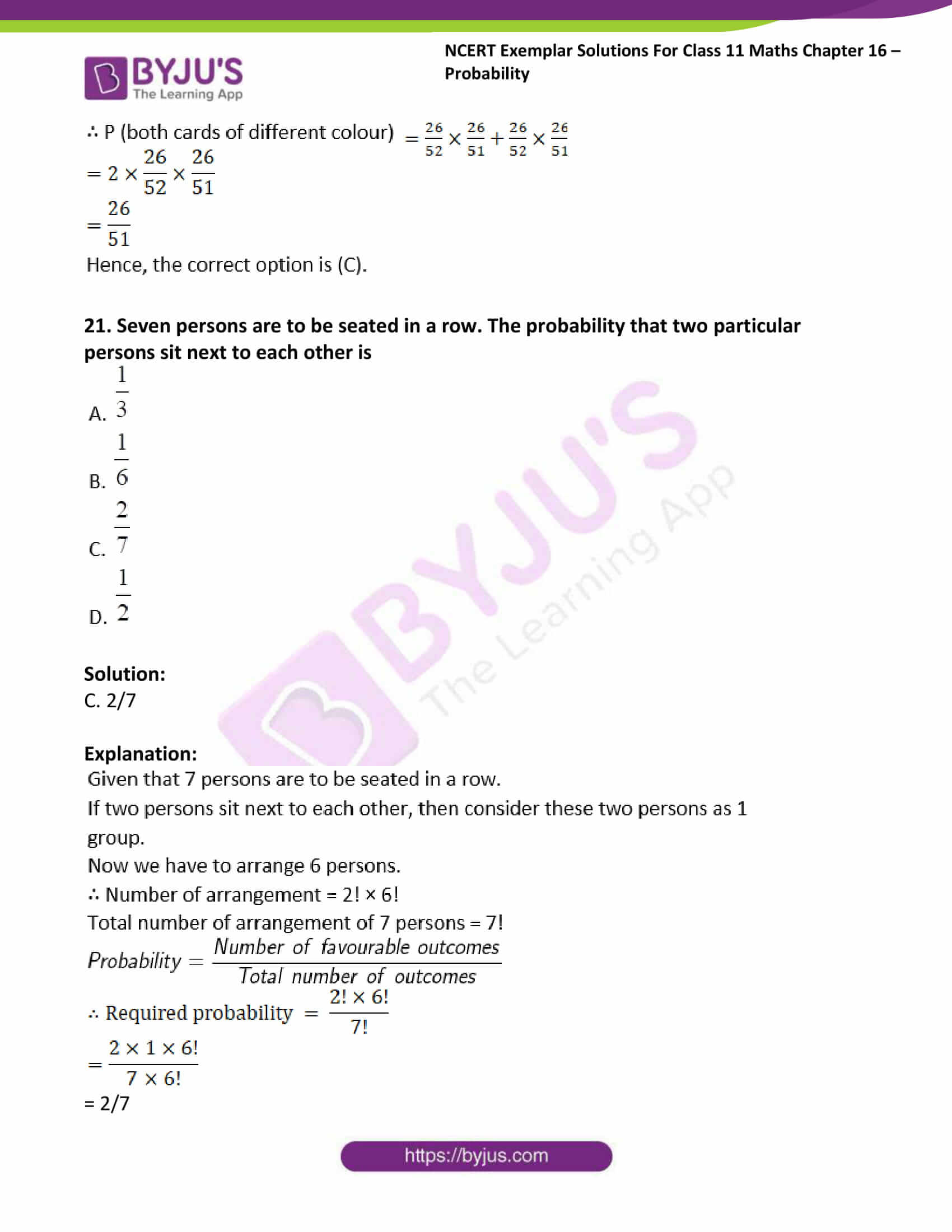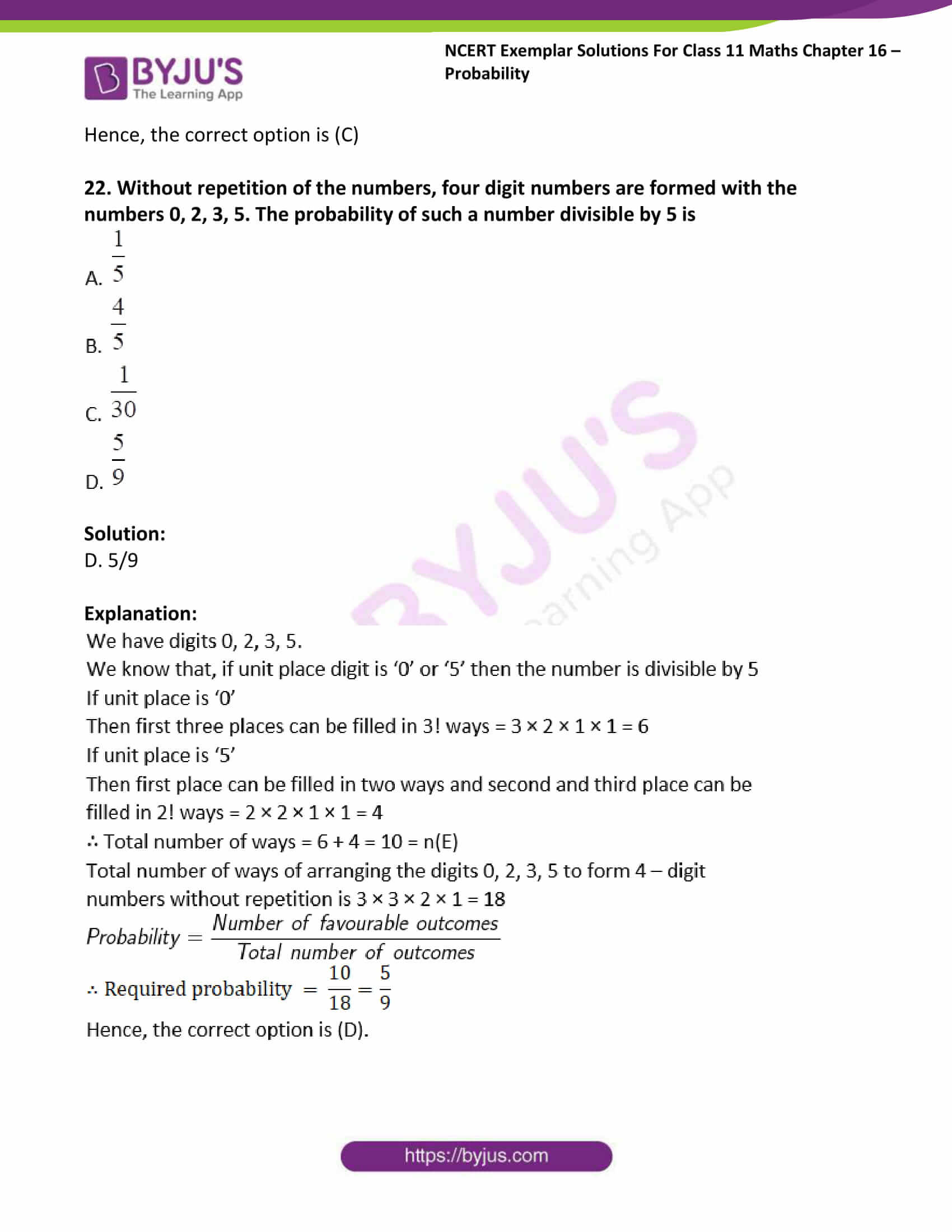### Access answers to Maths NCERT Exemplar Solutions For Class 11 Chapter 16 – Probability

Exercise Page no: 296

1. If the letters of the word ALGORITHM are arranged at random in a row what is the probability the letters GOR must remain together as a unit?

Solution: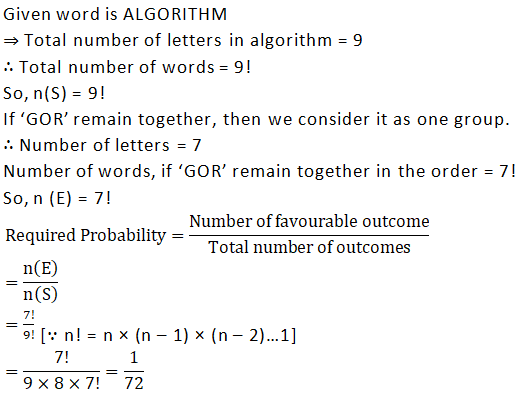2. Six new employees, two of whom are married to each other, are to be assigned six desks that are lined up in a row. If the assignment of employees to desks is made randomly, what is the probability that the married couple will have nonadjacent desks?

Solution:

Total new employees = 6

So, they can be arranged in 6! Ways

∴ n (S) = 6! = 6 × 5 × 4 × 3 × 2 × 1 = 720

Two adjacent desks for married couple can be selected in 5 ways i.e. (1, 2), (2, 3), (3, 4), (4, 5), (5, 6)

Married couple can be arranged in the two desks in 2! Ways

Other four persons can be arranged in 4! Ways

So, number of ways in which married couple occupy adjacent desks

= 5 × 2! × 4!

= 5 × 2 × 1 × 4 × 3 × 2 × 1

= 240

So, number of ways in which married couple occupy non – adjacent desks = 6! – 240

= (6 × 5 × 4 × 3 × 2 × 1) – 240

= 720 – 240

= 480 = n (E)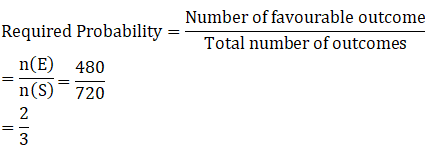3. Suppose an integer from 1 through 1000 is chosen at random, find the probability that the integer is a multiple of 2 or a multiple of 9.

Solution:

We have integers 1, 2, 3 … 1000

∴ Total number of outcomes, n(S) = 1000

Number of integers which are multiple of 2 are 2, 4, 6, 8, 10, … 1000

Let p be the number of terms

We know that, ap = a + (p – 1) d

Here, a = 2, d = 2 and ap = 1000

Putting the value, we get

2 + (p – 1)2 = 1000

⇒ 2 + 2p – 2 = 1000

p = 1000/2

⇒ p = 500

Total number of integers which are multiple of 2 = 500

Let the number of integers which are multiple of 9 be n.

Number which are multiples of 9 are 9, 18, 27, …999

∴ nth term = 999

We know that, an = a + (n – 1) d

Here, a = 9, d = 9 and an = 999

Putting the value, we get

9 + (n – 1)9 = 999

⇒ 9 + 9n – 9 = 999

n = 999/9

⇒ n = 111

So, the number of multiples of 9 from 1 to 1000 is 111.

The multiple of 2 and 9 both are 18, 36, … 990.

Let m be the number of terms in above series.

∴ mth term = 990

We know that, am = a + (m – 1) d

Here, a = 9 and d = 9

Putting the value, we get

18 + (m – 1)18 = 990

⇒ 18 + 18m – 18 = 990

m = 990/18

⇒ m = 55

Number of multiples of 2 or 9

= No. of multiples of 2 + no. of multiples of 9 – No. of multiples of 2 and 9 both

= 500 + 111 – 55

= 556 = n (E)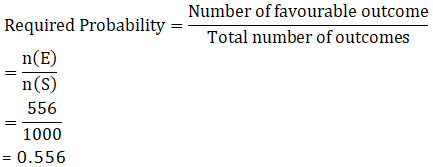4. An experiment consists of rolling a die until a 2 appears.
(i) How many elements of the sample space correspond to the event that the 2 appears on the kth roll of the die?
(ii) How many elements of the sample space correspond to the event that the 2 appears not later than the kth roll of the die?

Solution:

Given number of outcomes when die is thrown = 6

(i) Given that 2 appears on the kth roll of the die.

So, first (k – 1)th roll have 5 outcomes each and kth roll results 2

∴ Number of outcomes = 5k-1

(ii) If we consider that 2 appears not later than kth roll of the die, then 2 comes before kth roll.

If 2 appears in first roll, number of ways = 1 outcome

If 2 appears in second roll, number of ways = 5 x 1 (as first roll does not result in 2)

If 2 appears in third roll, number of ways = 5 x 5 x 1 (as first two rolls do not result in 2)

Similarly, if 2 appears in (k – 1)th roll, number of ways

= (5 x 5 x 5 … (k- 1) times) x 1

= 5k-1

Possible outcomes if 2 appears before kth roll

= 1 + 5 + 52 + 53+ … + 5k-1

Here, we get the series

We know that,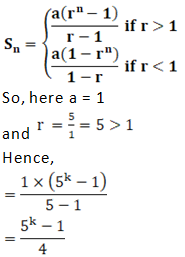5. A die is loaded in such a way that each odd number is twice as likely to occur as each even number. Find P(G), where G is the event that a number greater than 3 occurs on a single roll of the die.

Solution:

Given that probability of odd numbers

= 2 × (Probability of even number)

⇒ P (Odd) = 2 × P (Even)

Now, P (Odd) + P (Even) = 1

⇒ 2 P (Even) + P (Even) = 1

⇒ 3 P (Even) = 1

P (Even) = 1/3

So,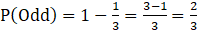Now, Total number occurs on a single roll of die = 6

And the number greater than 3 = 4, 5 or 6

So, P (G) = P (number greater than 3)

= P (number is 4, 5 or 6)

Here, 4 and 6 are even numbers and 5 is odd

∴ P (G) = 2 × P (Even) × P (Odd)

= 2 × 1/3 × 2/3

= 4/9

Hence, the required probability is 4/9

6. In a large metropolitan area, the probabilities are .87, .36, .30 that a family (randomly chosen for a sample survey) owns a colour television set, a black and white television set, or both kinds of sets. What is the probability that a family owns either anyone or both kinds of sets?

Solution:

E1 = Event that a family owns colour television

E2 = Event that the family owns black and white television

Given that P (E1) = 0.87

P (E2) = 0.36 and P (E1 ⋂ E2) = 0.30

Now, we have to find the probability that a family owns either anyone or both kinds of sets.

By General Addition Rule, we have

P (A ∪ B) = P(A) + P(B) – P (A ∩ B)

∴ P (E1⋃ E2) = P (E1) + P (E2) – P (E1⋂ E2)

= 0.87 + 0.36 – 0.30

= 1.23 – 0.30

= 0.93

Hence, the required probability is 0.93

7. If A and B are mutually exclusive events, P (A) = 0.35 and P (B) = 0.45, find
(a) P (A′)
(b) P (B′)
(c) P (A ∪ B)
(d) P (A ∩ B)
(e) P (A ∩ B′)
(f) P (A′∩ B′)

Solution:

Given that P (A) = 0.35 and P (B) = 0.45

∵ The events A and B are mutually exclusive then P (A ⋂ B) = 0

(a) To find (a) P (A′)

We know that,

P (A) + P (A’) = 1

⇒ 0.35 + P(A’) = 1 [given]

⇒ P (A’) = 1 – 0.35

⇒ P (A’) = 0.65

(b) To find (b) P (B′)

We know that,

P (B) + P (B’) = 1

⇒ 0.45 + P (B’) = 1

⇒ P (B’) = 1 – 0.45

⇒ P (B’) = 0.55

(c) To find (c) P (A ⋃ B)

We know that,

P (A ∪ B) = P (A) + P (B) – P (A ∩ B)

⇒ P (A ⋃ B) = 0.35 + 0.45 – 0 [given]

⇒ P (A ⋃ B) = 0.80

(d) To find (d) P (A ⋂ B)

It is given that A and B are mutually exclusive events.

∴ P (A ⋂ B) = 0

(e) To find (e) P (A ⋂ B’)

P (A ⋂ B’) = P (A) – P (A ⋂ B)

= 0.35 – 0

= 0.35

(f) To find (f) P (A’ ⋂ B’)

P (A’ ⋂ B’) = P (A ⋃ B)’

= 1 – P (A ⋃ B)

= 1 – 0.8 [from part (c)]

= 0.2

8. A team of medical students doing their internship have to assist during surgeries at a city hospital. The probabilities of surgeries rated as very complex, complex, routine, simple or very simple are respectively, 0.15, 0.20, 0.31, 0.26, .08. Find the probabilities that a particular surgery will be rated.
(a) complex or very complex;
(b) neither very complex nor very simple;
(c) routine or complex
(d) routine or simple

Solution:

Let

E1 = event that surgeries are rated as very complex

E2 = event that surgeries are rated as complex

E3 = event that surgeries are rated as routine

E4 = event that surgeries are rated as simple

E5 = event that surgeries are rated as very simple

Given: P (E1) = 0.15, P (E2) = 0.20, P (E3) = 0.31, P (E4) = 0.26, P (E5) = 0.08

(a) P (complex or very complex) = P (E1 or E2) = P (E1⋃ E2)

P (A ∪ B) = P(A) + P(B) – P (A ∩ B)

⇒ P (E1⋃ E2) = P (E1) + P (E2) – P (E1⋂ E2)

= 0.15 + 0.20 – 0 [given] [∵ All events are independent]

= 0.35

(b) P (neither very complex nor very simple) = P (E1’ ⋂ E5’)

= P (E1⋃ E5)’

= 1 – P (E1⋃ E5)

[∵By Complement Rule]

= 1 – [P (E1) + P (E5) – P (E1⋂ E5)] [∵ By General Addition Rule]

= 1 – [0.15 + 0.08 – 0]

= 1 – 0.23

= 0.77

(c) P (routine or complex) = P (E3⋃ E2)

= P (E3) + P (E2) – P (E3⋂ E2)

= 0.31 + 0.20 – 0 [given]

= 0.51

(d) P (routine or simple) = P (E3⋃ E4)

= P (E3) + P (E4) – P (E3⋂ E4)

= 0.31 + 0.26 – 0 [given]

= 0.57

9. Four candidates A, B, C, D have applied for the assignment to coach a school cricket team. If A is twice as likely to be selected as B, and B and C are given about the same chance of being selected, while C is twice as likely to be selected as D, what are the probabilities that
(a) C will be selected?
(b) A will not be selected?

Solution:

Given that A is twice as likely to be selected as B

i.e. P (A) = 2 P (B) ……1

and C is twice as likely to be selected as D

i.e. P (C) = 2 P (D) ……2

Now, B and C are given about the same chance

∴ P (B) = P (C) ……3

Since, sum of all probabilities = 1

∴ P (A) + P (B) + P (C) + P (D) = 1

⇒ P (A) + P (B) + P (B) + P (D) = 1 [from 3]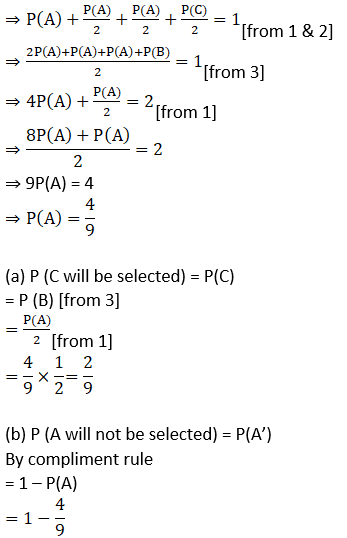= 5/9

10. One of the four persons John, Rita, Aslam or Gurpreet will be promoted next month. Consequently, the sample space consists of four elementary outcomes S = {John promoted, Rita promoted, Aslam promoted, Gurpreet promoted} You are told that the chances of John’s promotion is same as that of Gurpreet, Rita’s chances of promotion are twice as likely as Johns. Aslam’s chances are four times that of John.
(a) Determine P (John promoted)
P (Rita promoted)
P (Aslam promoted)
P (Gurpreet promoted)
(b) If A = {John promoted or Gurpreet promoted}, find P (A).

Solution:

Given Sample Space, S = John promoted, Rita promoted, Aslam promoted, Gurpreet promoted

Let E1 = events that John promoted

E2 = events that Rita promoted

E3 = events that Aslam promoted

E4 = events that Gurpreet promoted

It is given that chances of John’s promotion is same as that of Gurpreet

P (E1) = P (E4) ……1

It is given that Rita’s chances of promotion are twice as likely as John

P (E2) = 2P (E1) ……2

and Aslam’s chances of promotion are four times that of John

P (E3) = 4 P (E1) ……3

Since, sum of all probabilities = 1

⇒ P (E1) + P (E2) + P (E3) + P (E4) = 1

⇒ P (E1) + 2P (E1) + 4 P (E1) + P (E1) = 1

⇒ 8P (E1) = 1

⇒ P (E1) = 1/8 ….4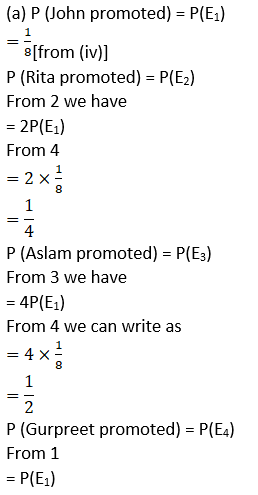= ½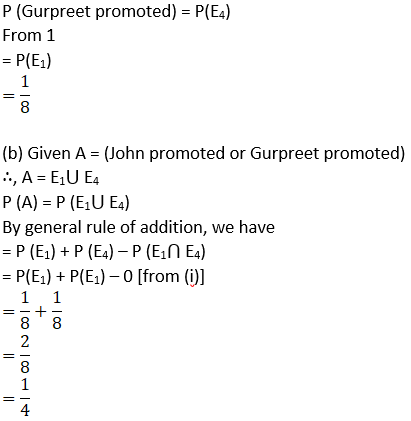11. The accompanying Venn diagram shows three events, A, B, and C, and also the probabilities of the various intersections (for instance, P (A ∩ B) = .07. Determine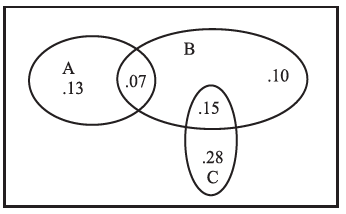(a) P (A)
(b)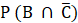(c) P (A ∪ B)
(d) P (A ∩ B)
(e) P (B ∩ C)
(f) Probability of exactly one of the three occurs.

Solution:

Given P (A ⋂ B) = 0.07

From the given Venn Diagram

(a) P(A)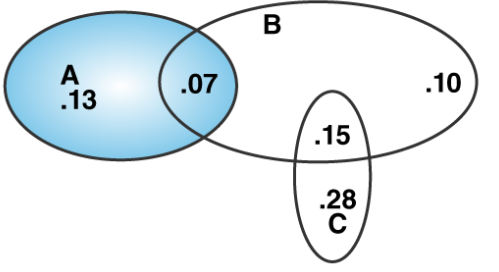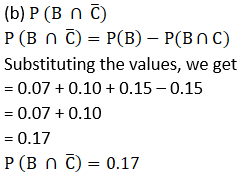(c) P (A ⋃ B)

P (A ⋃ B) = P (A) + P (B) – P (A ⋂ B)

Now substituting the values, we get

⇒ P (A ⋃ B) = 0.20 + (0.07 + 0.10 + 0.15) – 0.07

⇒ P (A ⋃ B) = 0.20 + 0.25

⇒ P (A ⋃ B) = 0.45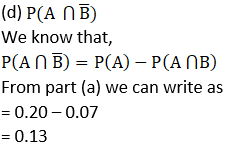(e) P (B ⋂ C)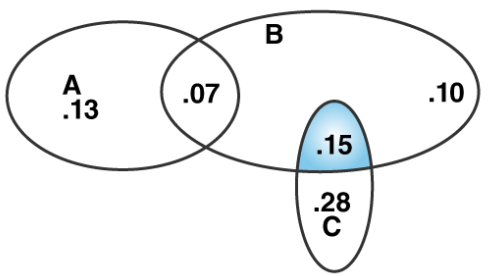From the Venn diagram we have

P (B ⋂ C) = 0.15

(f) Probability of exactly one of the three occurs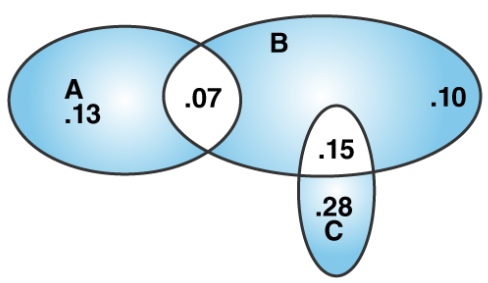P (exactly one of the three occurs) = 0.13 + 0.10 + 0.28

= 0.51

12. One urn contains two black balls (labelled B1 and B2) and one white ball. A second urn contains one black ball and two white balls (labelled W1 and W2). Suppose the following experiment is performed. One of the two urns is chosen at random. Next a ball is randomly chosen from the urn. Then a second ball is chosen at random from the same urn without replacing the first ball.
(a) Write the sample space showing all possible outcomes
(b) What is the probability that two black balls are chosen?
(c) What is the probability that two balls of opposite colour are chosen?

Solution:

Given that one urn contains two black balls and one white ball

and second urn contains one black ball and two white balls.

It is also given that one of the two urns is chosen, then a ball is randomly chosen from the urn, then second ball is chosen at random from the same urn without replacing the first ball

(a) Sample Space S = {B1B2, B1W, B2W, B2B1, WB1, WB2, W1W2, W1B, W2B, W2W1, BW1, BW2}

Total number of sample space = 12

(b) If two black balls are chosen

Total outcomes = 12

Favourable outcomes are B1B2, B2B1

∴ Total favourable outcomes = 2

We know that,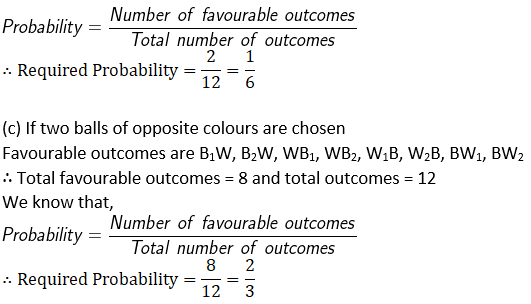13. A bag contains 8 red and 5 white balls. Three balls are drawn at random. Find the Probability that

(a) All the three balls are white
(b) All the three balls are red
(c) One ball is red and two balls are white

Solution:

Given that, number of red balls = 8

Number of white balls = 5

∴ Total balls, n = 13

It is given that 3 balls are drawn at random

⇒ r = 3

∴ n(S) = nCr = 13C3

(a) All the three balls are white

We know that,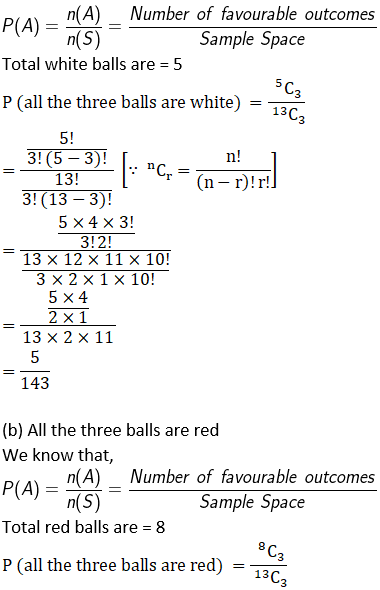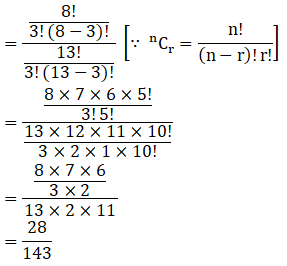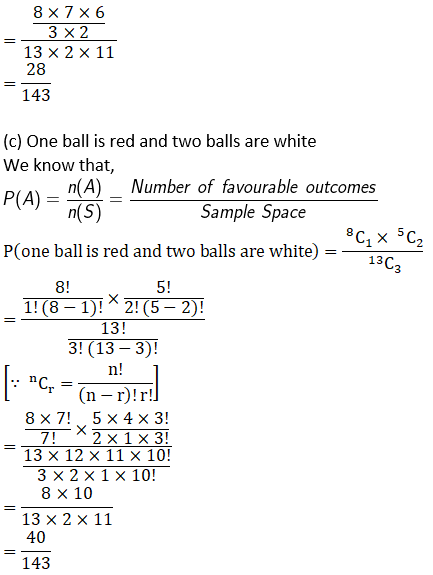14. If the letters of the word ASSASSINATION are arranged at random. Find the Probability that
(a) Four S’s come consecutively in the word
(b) Two I’s and two N’s come together
(c) All A’s are not coming together
(d) No two A’s are coming together.

Solution:

Given word is ASSASSINATION

Total number of letters in ASSASSINATION is 13

In word ASSASSINATION, there are 3A’s, 4S’s, 2I’s, 2N’s, 1T’s and 1O’s

Total number of ways these letters can be arranged =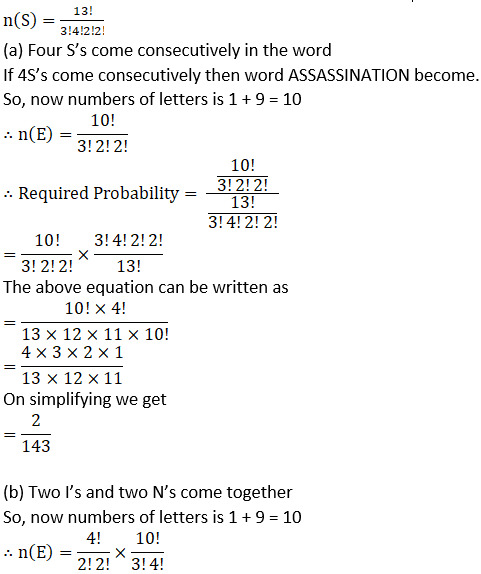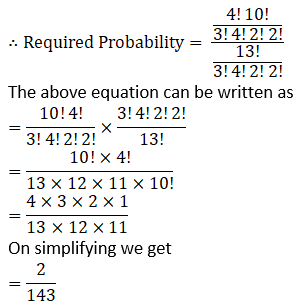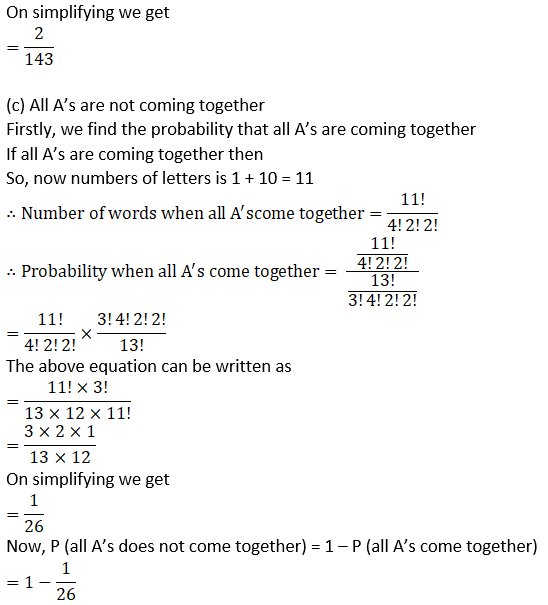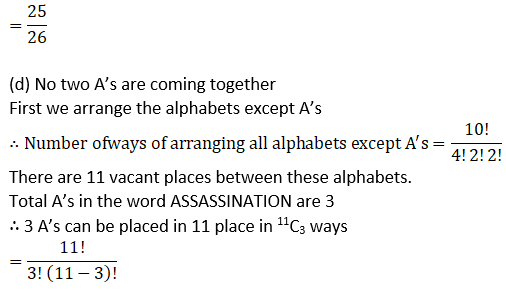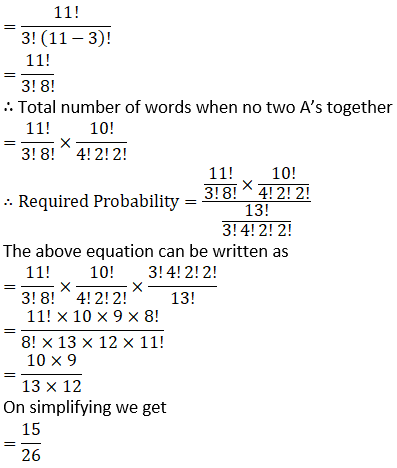15. A card is drawn from a deck of 52 cards. Find the probability of getting a king or a heart or a red card.

Solution: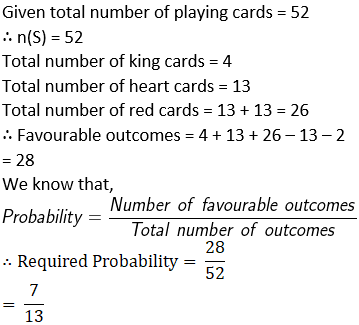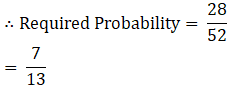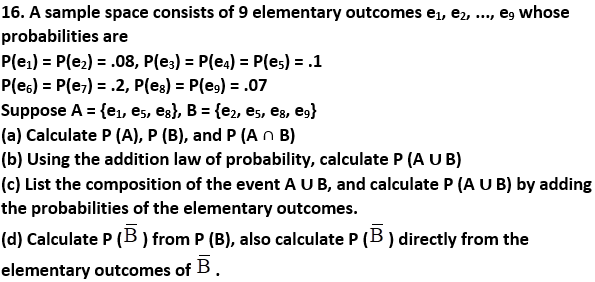Solution:

Given

S = {e1, e2, e3, e4, e5, e6, e7, e8, e9}

A = {e1, e5, e8} and B = {e2, e5, e8, e9}

P(e1) = P(e2) = .08, P(e3) = P(e4) = P(e5) = .1

P(e6) = P(e7) = .2, P(e8) = P(e9) = .07

(a) To find P (A), P (B) and P (A ⋂ B)

A = {e1, e5, e8}

P (A) = P (e1) + P (e5) + P (e8)

Substituting the values, we get

⇒ P (A) = 0.08 + 0.1 + 0.07

⇒ P (A) = 0.25

B = {e2, e5, e8, e9}

P (B) = P (e2) + P (e5) + P (e8) + P (e9)

Substituting the values, we get

⇒ P (B) = 0.08 + 0.1 + 0.07 + 0.07 [given]

⇒ P (B) = 0.32

Now, we have to find P (A ⋂ B)

A = {e1, e5, e8} and B = {e2, e5, e8, e9}

∴ A ⋂ B = {e5, e8}

⇒ P (A ⋂ B) = P (e5) + P (e8)

= 0.1 + 0.07

= 0.17

(b) To find P (A ⋃ B)

P (A ⋃ B) = P (A) + P (B) – P (A ⋂ B)

from part (a), we have

P (A) = 0.25, P (B) = 0.32 and P (A ⋂ B) = 0.17

Putting the values, we get

P (A ⋃ B) = 0.25 + 0.32 – 0.17

= 0.40

(c) A = {e1, e5, e8} and B = {e2, e5, e8, e9}

∴ A ⋃ B = {e1, e2, e5, e8, e9}

⇒ P (A ⋃ B) = P (e1) + P (e2) + P (e5) + P (e8) + P (e9)

Substituting the values, we get

= 0.08 +0.08 + 0.1 + 0.07 + 0.07

= 0.40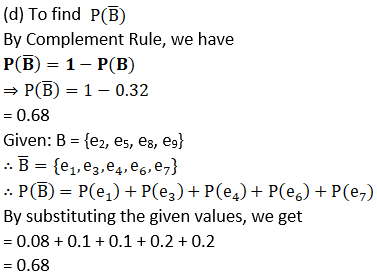17. Determine the probability p, for each of the following events.
(a) An odd number appears in a single toss of a fair die.
(b) At least one head appears in two tosses of a fair coin.
(c) A king, 9 of hearts, or 3 of spades appears in drawing a single card from a well shuffled ordinary deck of 52 cards.
(d) The sum of 6 appears in a single toss of a pair of fair dice.

Solution: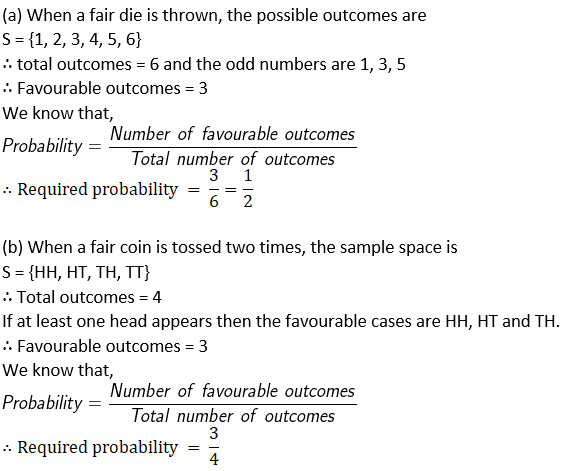(c) When a pair of dice is rolled, total number of cases

S = {(1,1), (1,2), (1,3), (1,4), (1,5), (1,6)

(2,1), (2,2), (2,3), (2,4), (2,5), (2,6)

(3,1), (3,2), (3,3), (3,4), (3,5), (3,6)

(4,1), (4,2), (4,3), (4,4), (4,5), (4,6)

(5,1), (5,2), (5,3), (5,4), (5,5), (5,6)

(6,1), (6,2), (6,3), (6,4), (6,5), (6,6)}

Total Sample Space, n(S) = 36

If sum is 6 then possible outcomes are (1,5), (2,4), (3,3), (4,2) and (5,1).

∴ Favourable outcomes = 5

We know that,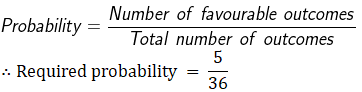objective TYPE questions:

Choose the correct answer out of four given options in each of the Exercises 18 to 29 (M.C.Q.).

18. In a non-leap year, the probability of having 53 Tuesdays or 53 Wednesdays is
A. 1/7
B. 2/7
C. 3/7
D. none of these

Solution:

B. 2/7

Explanation:

We know that in a non-leap year, there are 365 days and we know that there are 7 days in a week

∴ 365 ÷ 7 = 52 weeks + 1 day

This 1 day can be Sunday, Monday, Tuesday, Wednesday, Thursday, Friday, Saturday

∴ Total Outcomes = 7

If this day is a Tuesday or Wednesday, then the year will have 53 Tuesday or 53 Wednesday.

∴P (non-leap year has 53 Tuesdays or 53 Wednesdays) = 1/7 + 1/7 = 2/7

Hence, the correct option is (B).

19. Three numbers are chosen from 1 to 20. Find the probability that they are not consecutive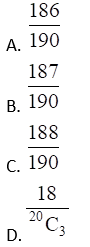Solution:

B. 187/190

Explanation:

Since, the set of three consecutive numbers from 1 to 20 are (1, 2, 3), (2, 3, 4), (3, 4, 5), …, (18,19,20)

Considering 3 numbers as a single digit

∴ the numbers will be 18

Now, we have to choose 3 numbers out of 20. This can be done in 20C3 ways

∴ n(S) = 20C3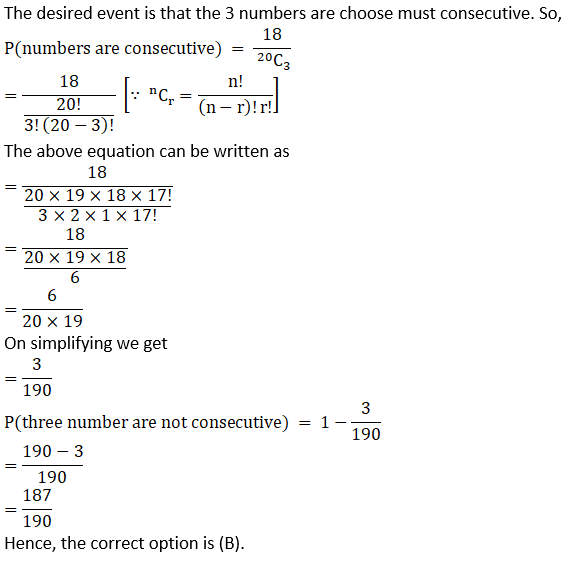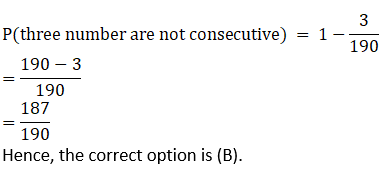20. While shuffling a pack of 52 playing cards, 2 are accidentally dropped. Find the probability that the missing cards to be of different colours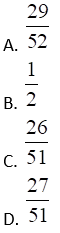Solution:

C. 26/51

Explanation: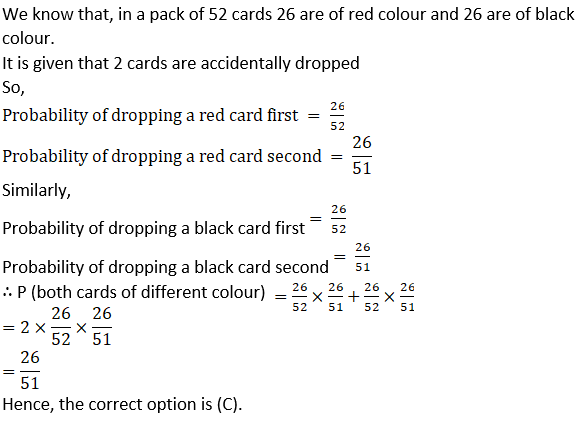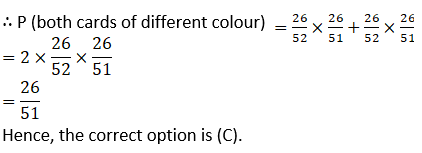21. Seven persons are to be seated in a row. The probability that two particular persons sit next to each other is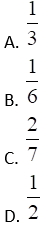Solution:

C. 2/7

Explanation: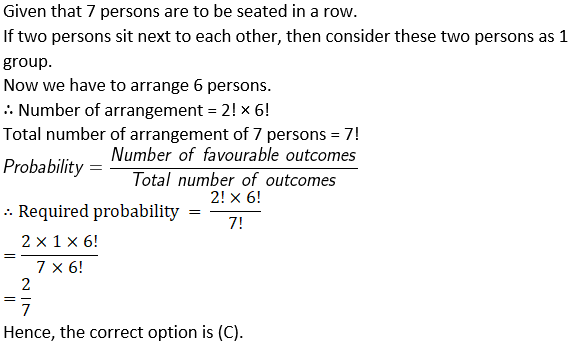= 2/7

Hence, the correct option is (C)

22. Without repetition of the numbers, four digit numbers are formed with the numbers 0, 2, 3, 5. The probability of such a number divisible by 5 is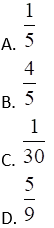Solution:

D. 5/9

Explanation: